FreeRDP
wlog.c File Reference
`#include <winpr/config.h>`
`#include <stdio.h>`
`#include <stdarg.h>`
`#include <string.h>`
`#include <winpr/crt.h>`
`#include <winpr/assert.h>`
`#include <winpr/print.h>`
`#include <winpr/debug.h>`
`#include <winpr/environment.h>`
`#include <winpr/wlog.h>`
`#include "wlog.h"`

## Macros

#define WLOG_FILTER_NOT_FILTERED   -1

#define WLOG_FILTER_NOT_INITIALIZED   -2

## Functions

static wLog * WLog_New (LPCSTR name, wLog *rootLogger)

static void WLog_Free (wLog *log)

static LONG WLog_GetFilterLogLevel (wLog *log)

static int WLog_ParseLogLevel (LPCSTR level)

static BOOL WLog_ParseFilter (wLog *root, wLogFilter *filter, LPCSTR name)

static BOOL WLog_ParseFilters (wLog *root)

static wLog * WLog_Get_int (wLog *root, LPCSTR name)

static void WLog_Uninit_ (void)

static void WLog_Lock (wLog *log)

static void WLog_Unlock (wLog *log)

static BOOL CALLBACK WLog_InitializeRoot (PINIT_ONCE InitOnce, PVOID Parameter, PVOID *Context)

static BOOL log_recursion (LPCSTR file, LPCSTR fkt, size_t line)

static BOOL WLog_Write (wLog *log, wLogMessage *message)

static BOOL WLog_WriteData (wLog *log, wLogMessage *message)

static BOOL WLog_WriteImage (wLog *log, wLogMessage *message)

static BOOL WLog_WritePacket (wLog *log, wLogMessage *message)

BOOL WLog_PrintMessageVA (wLog *log, DWORD type, DWORD level, size_t line, const char *file, const char *function, va_list args)

BOOL WLog_PrintMessage (wLog *log, DWORD type, DWORD level, size_t line, const char *file, const char *function,...)

DWORD WLog_GetLogLevel (wLog *log)

BOOL WLog_IsLevelActive (wLog *_log, DWORD _log_level)

BOOL WLog_SetStringLogLevel (wLog *log, LPCSTR level)

static BOOL WLog_reset_log_filters (wLog *log)

static BOOL WLog_AddStringLogFilters_int (wLog *root, LPCSTR filter)

static BOOL WLog_UpdateInheritLevel (wLog *log, DWORD logLevel)

BOOL WLog_SetLogLevel (wLog *log, DWORD logLevel)

static BOOL WLog_ParseName (wLog *log, LPCSTR name)

wLog * WLog_GetRoot (void)

static BOOL WLog_AddChild (wLog *parent, wLog *child)

static wLog * WLog_FindChild (wLog *root, LPCSTR name)

wLog * WLog_Get (LPCSTR name)

BOOL WLog_SetContext (wLog *log, const char *(*fkt)(void *), void *context)
Set a custom context for a dynamic logger. This can be used to print a customized prefix, e.g. some session id for a specific context. More...

## Variables

LPCSTR WLOG_LEVELS  = { "TRACE", "DEBUG", "INFO", "WARN", "ERROR", "FATAL", "OFF" }

static DWORD g_FilterCount = 0

static wLogFilter * g_Filters = NULL

static wLog * g_RootLog = NULL

## ◆ WLOG_FILTER_NOT_FILTERED

 #define WLOG_FILTER_NOT_FILTERED   -1

## ◆ WLOG_FILTER_NOT_INITIALIZED

 #define WLOG_FILTER_NOT_INITIALIZED   -2

## ◆ log_recursion()

 static BOOL log_recursion ( LPCSTR file, LPCSTR fkt, size_t line )
static
Here is the call graph for this function: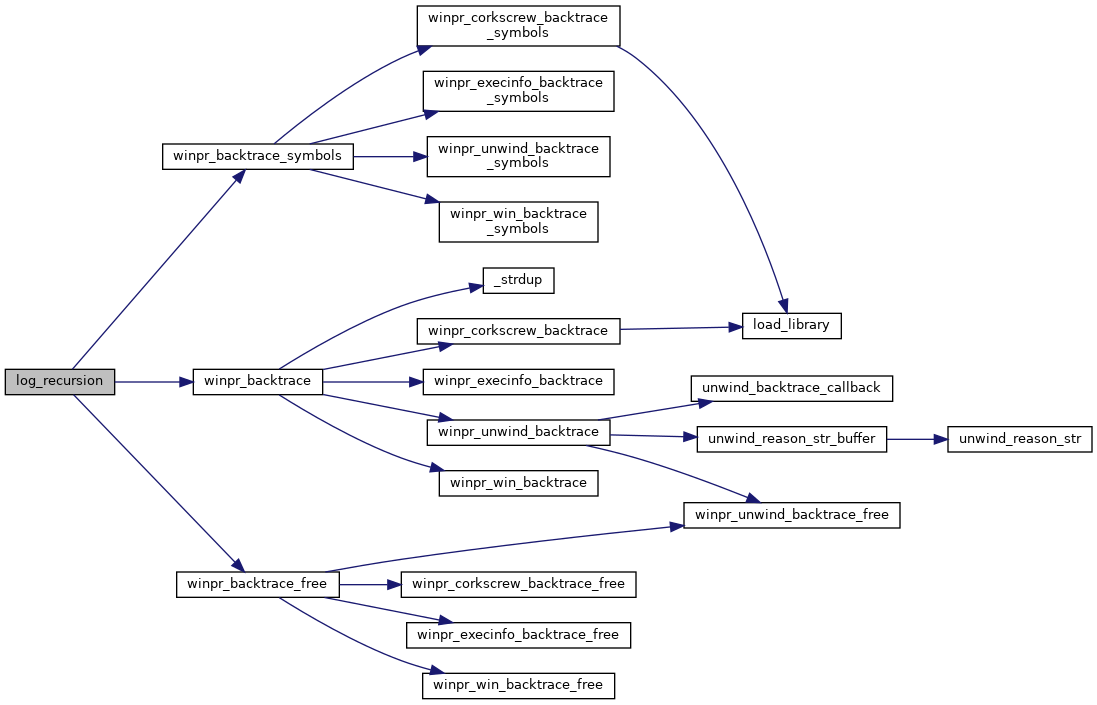Here is the caller graph for this function: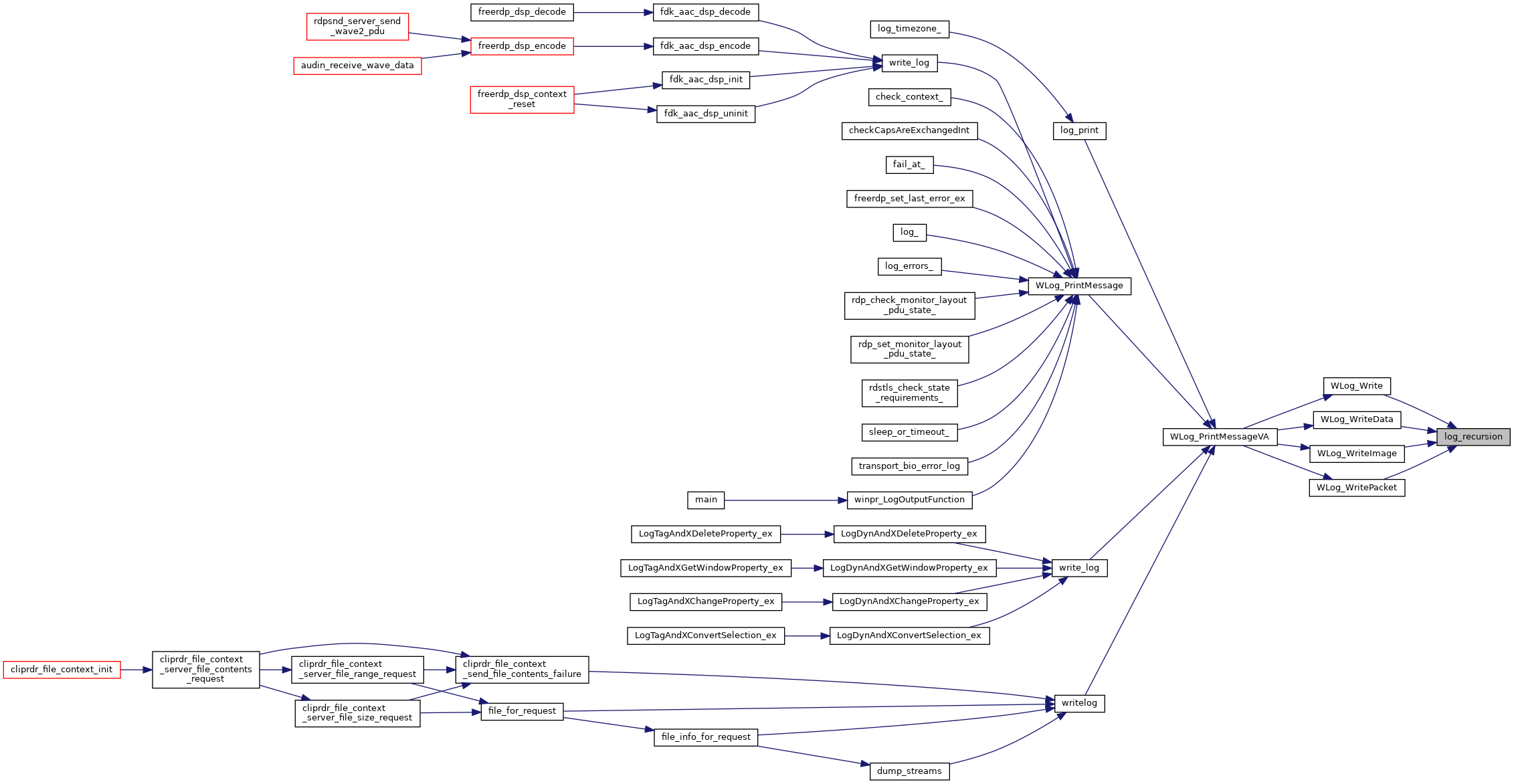static BOOL WLog_AddChild ( wLog * parent, wLog * child )
static
Here is the call graph for this function: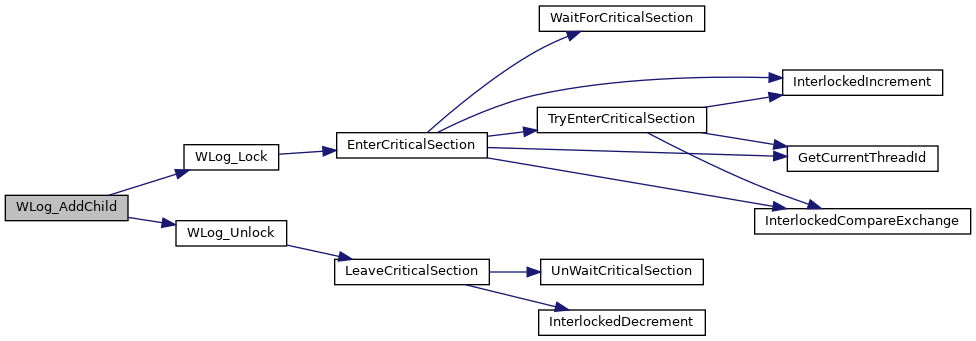Here is the caller graph for this function: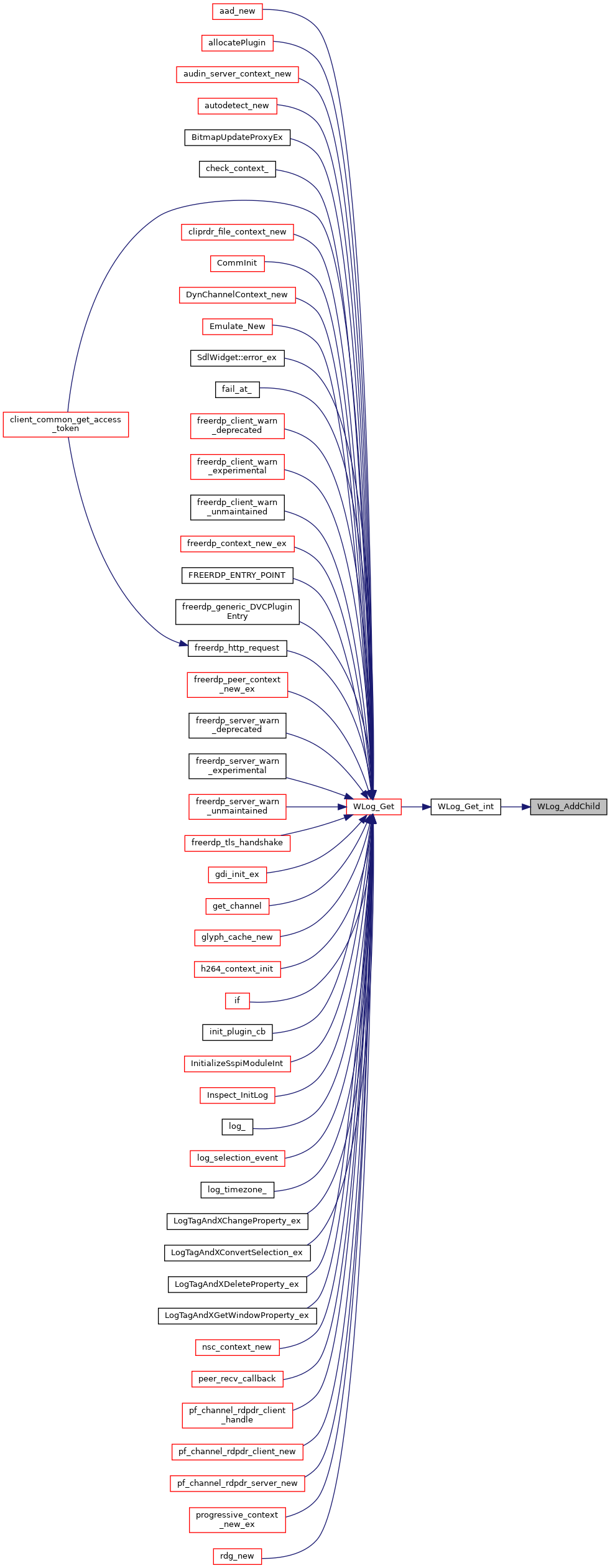BOOL WLog_AddStringLogFilters ( LPCSTR filter )
Here is the call graph for this function: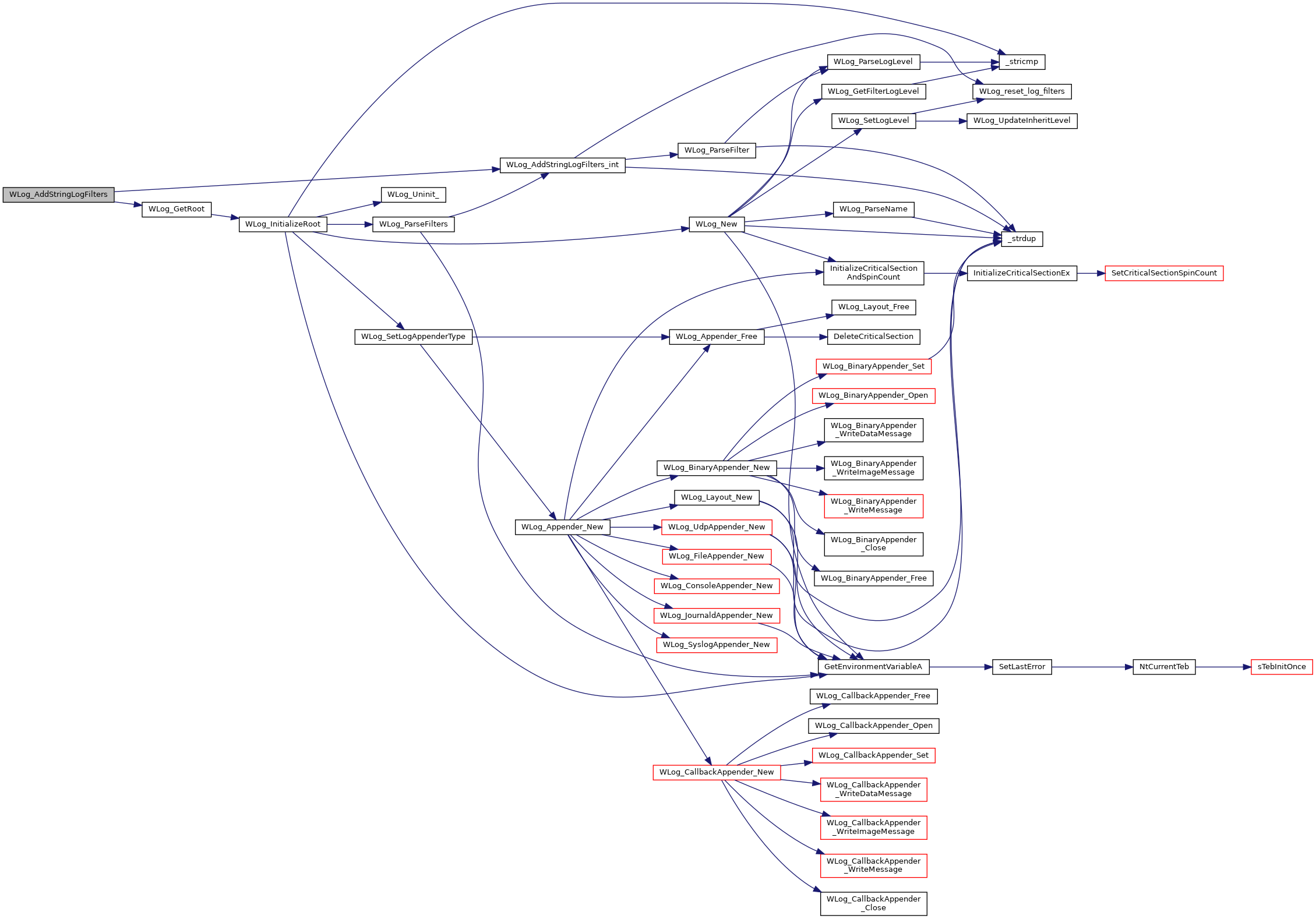Here is the caller graph for this function: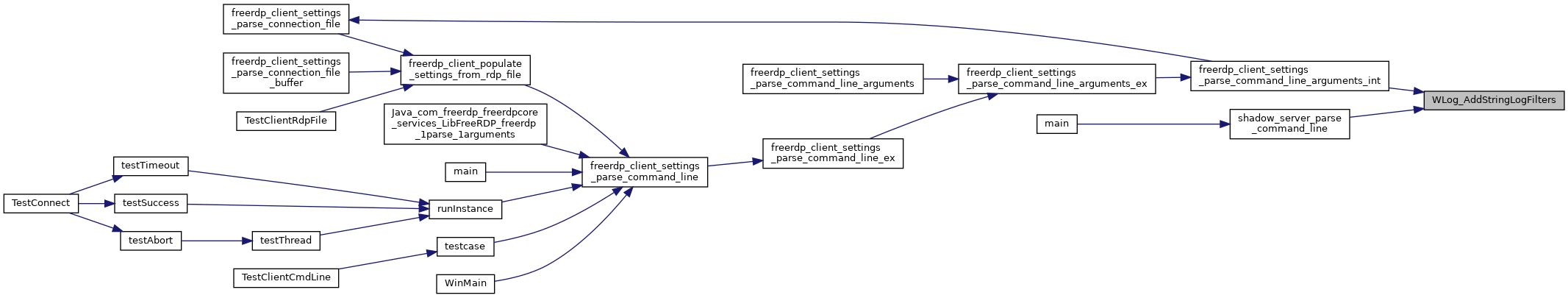static BOOL WLog_AddStringLogFilters_int ( wLog * root, LPCSTR filter )
static
Here is the call graph for this function: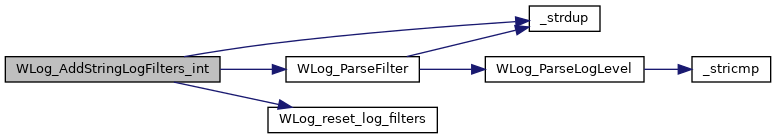Here is the caller graph for this function: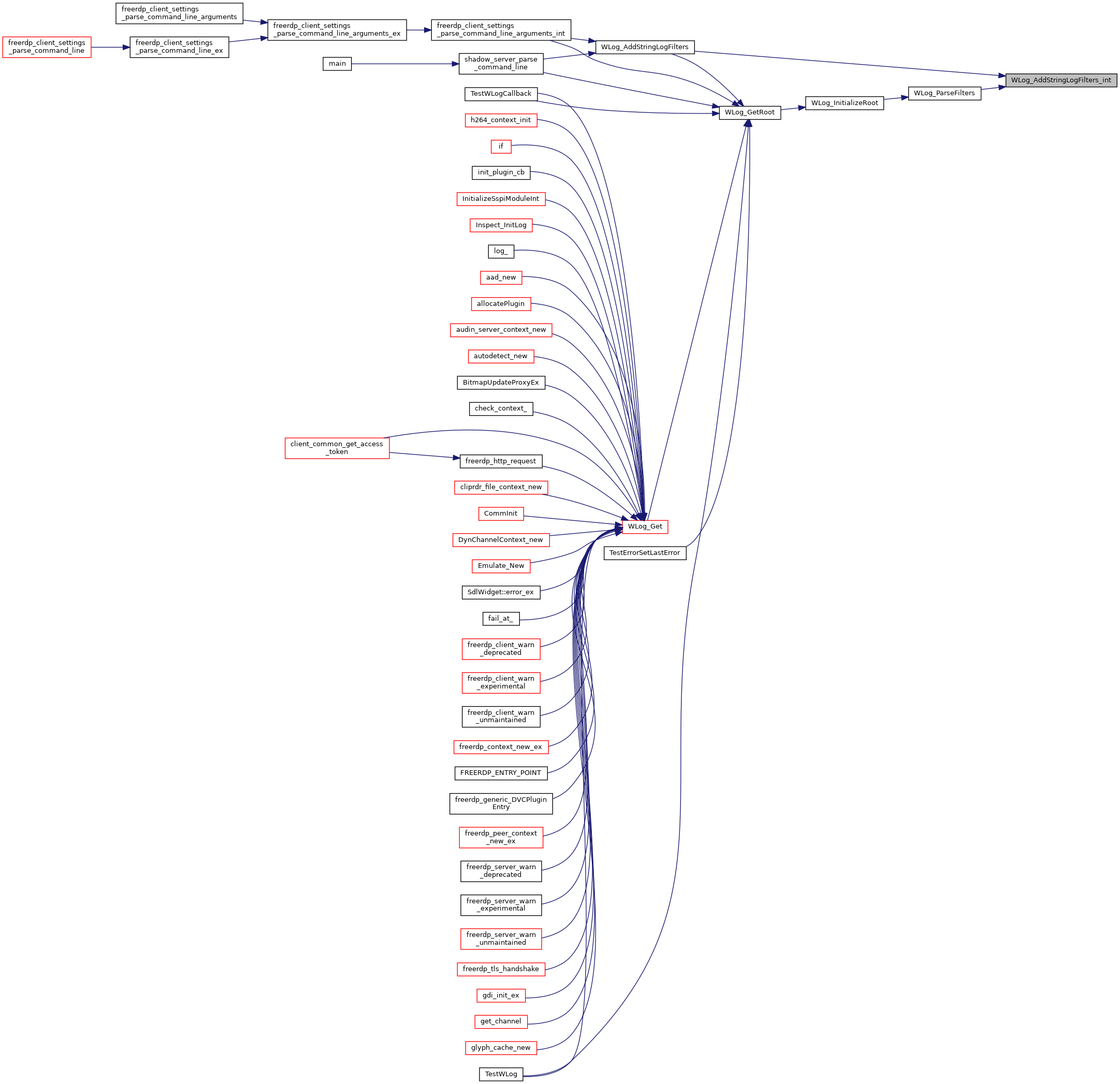## ◆ WLog_FindChild()

 static wLog* WLog_FindChild ( wLog * root, LPCSTR name )
static
Here is the call graph for this function: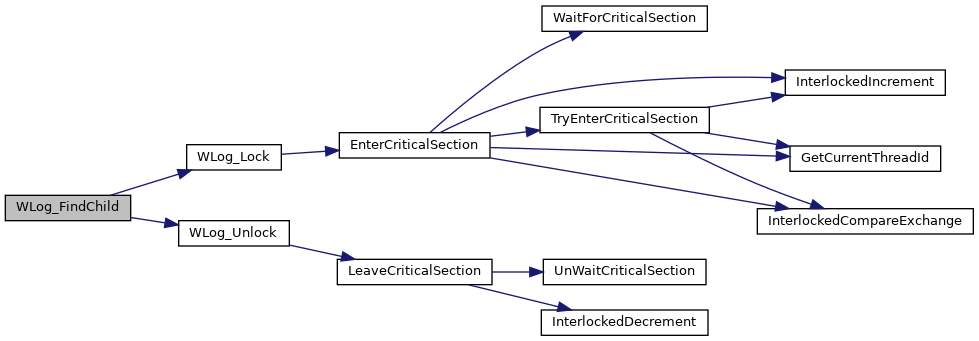Here is the caller graph for this function: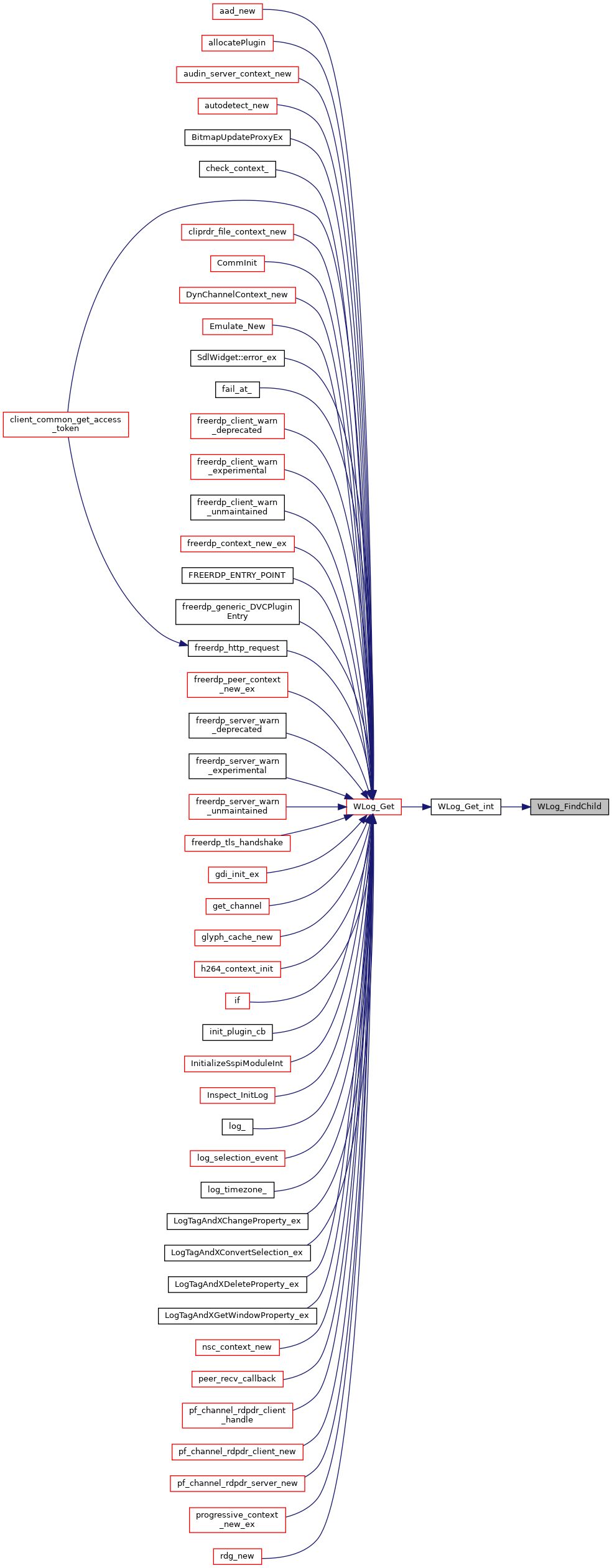## ◆ WLog_Free()

 void WLog_Free ( wLog * log )
static
Here is the call graph for this function:Here is the caller graph for this function: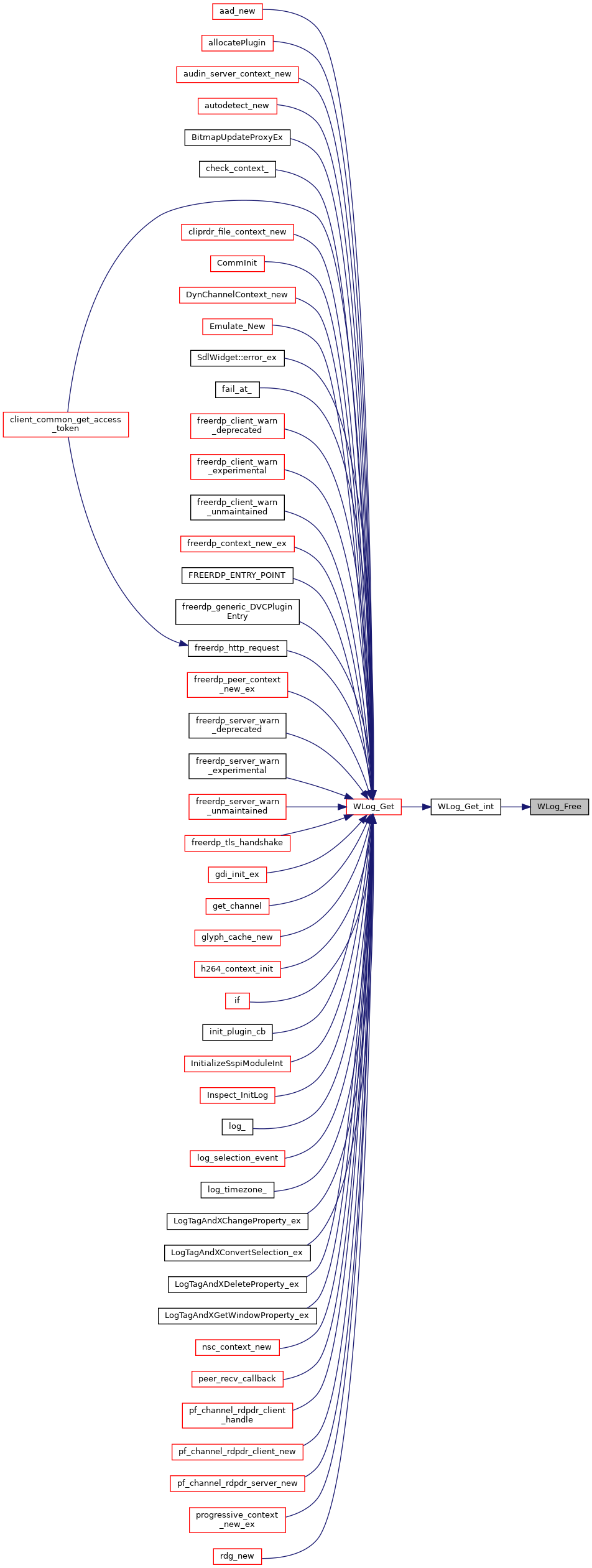## ◆ WLog_Get()

 wLog* WLog_Get ( LPCSTR name )
Here is the call graph for this function: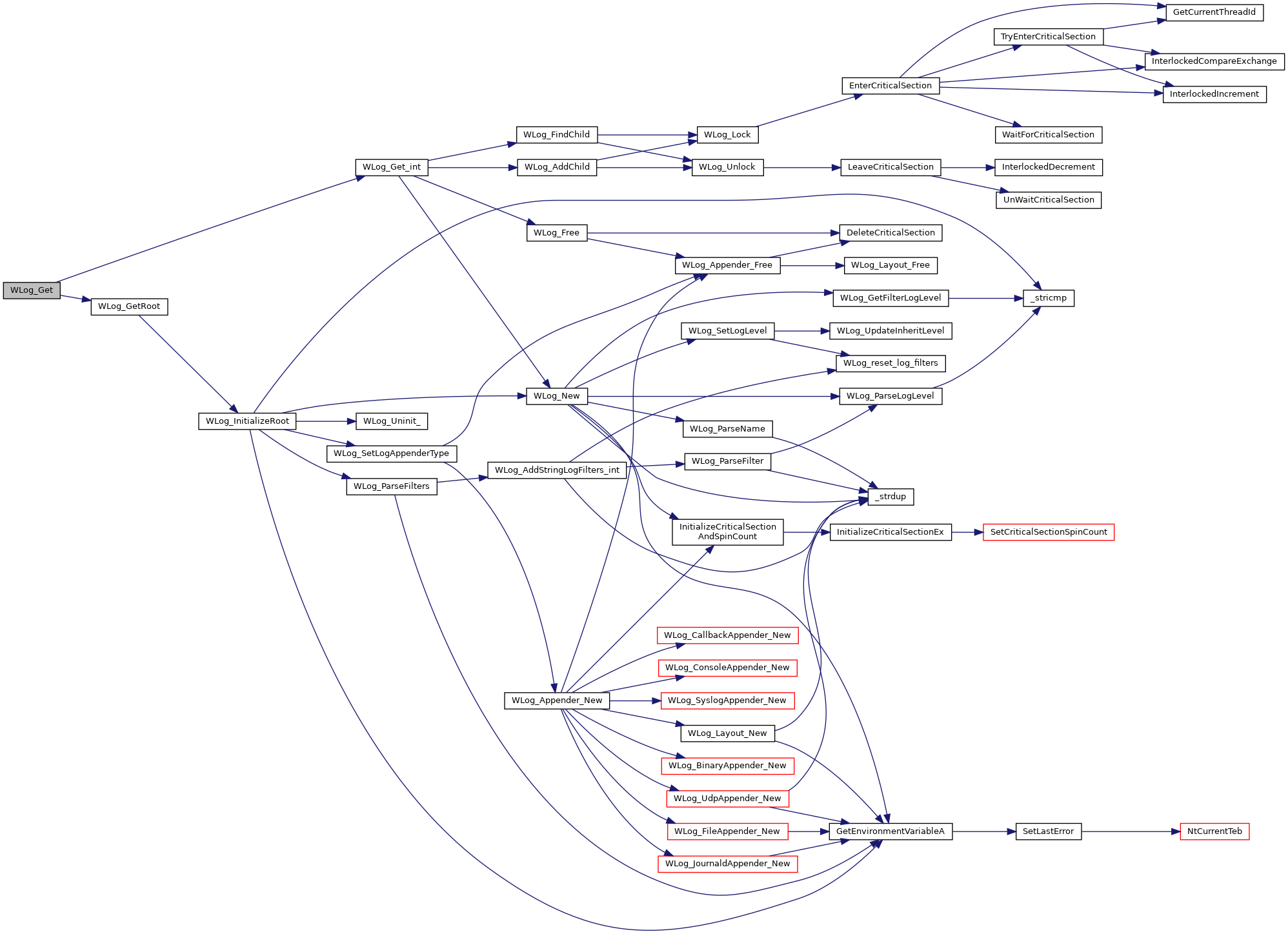## ◆ WLog_Get_int()

 static wLog * WLog_Get_int ( wLog * root, LPCSTR name )
static
Here is the call graph for this function: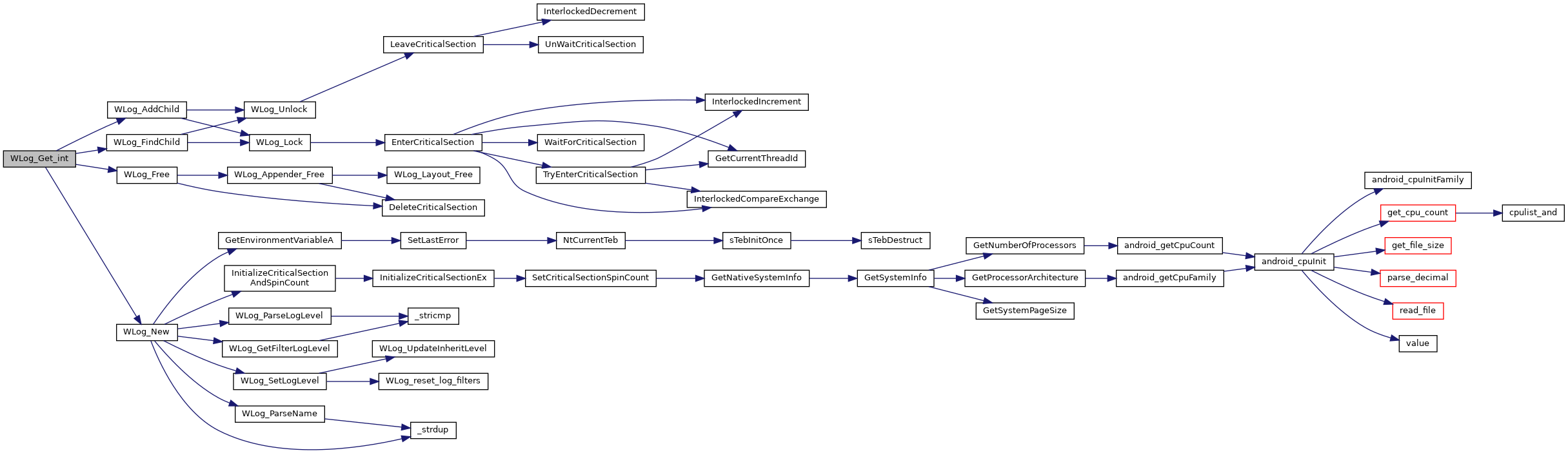Here is the caller graph for this function: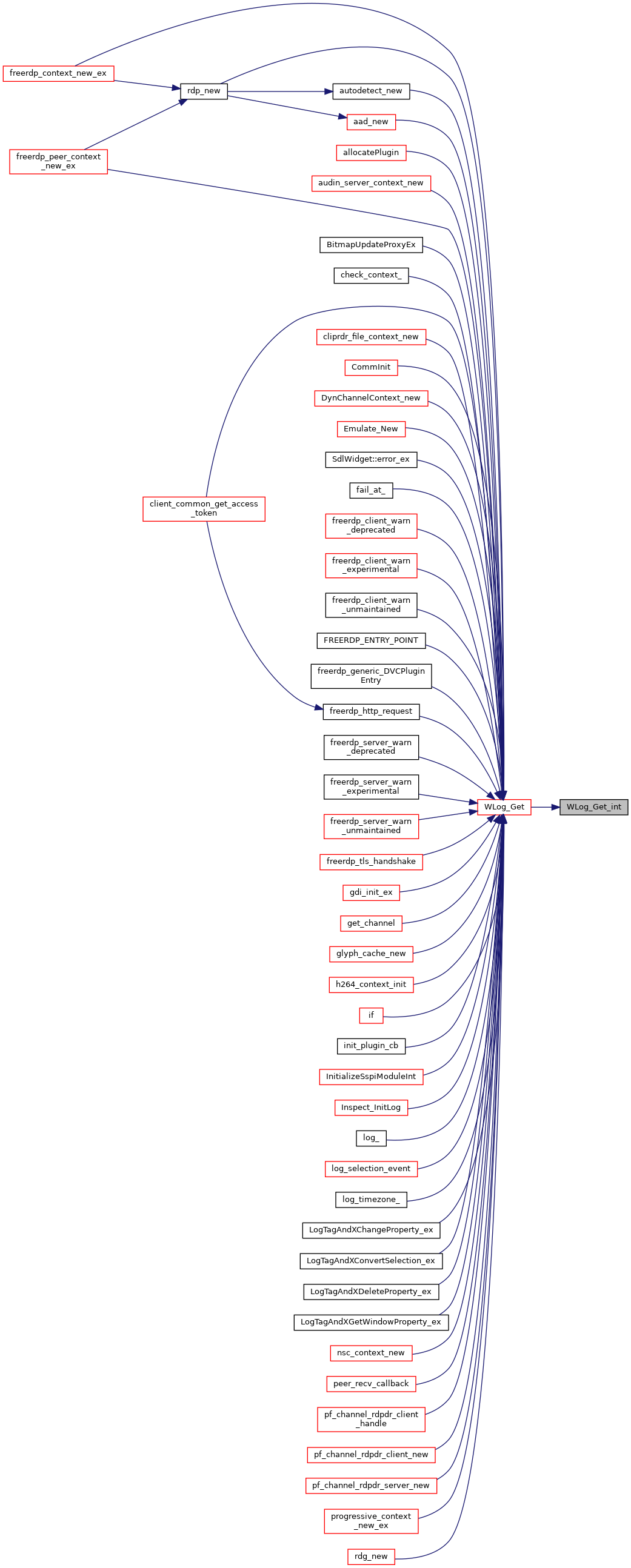## ◆ WLog_GetFilterLogLevel()

 LONG WLog_GetFilterLogLevel ( wLog * log )
static
Here is the call graph for this function: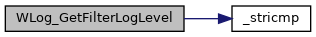Here is the caller graph for this function: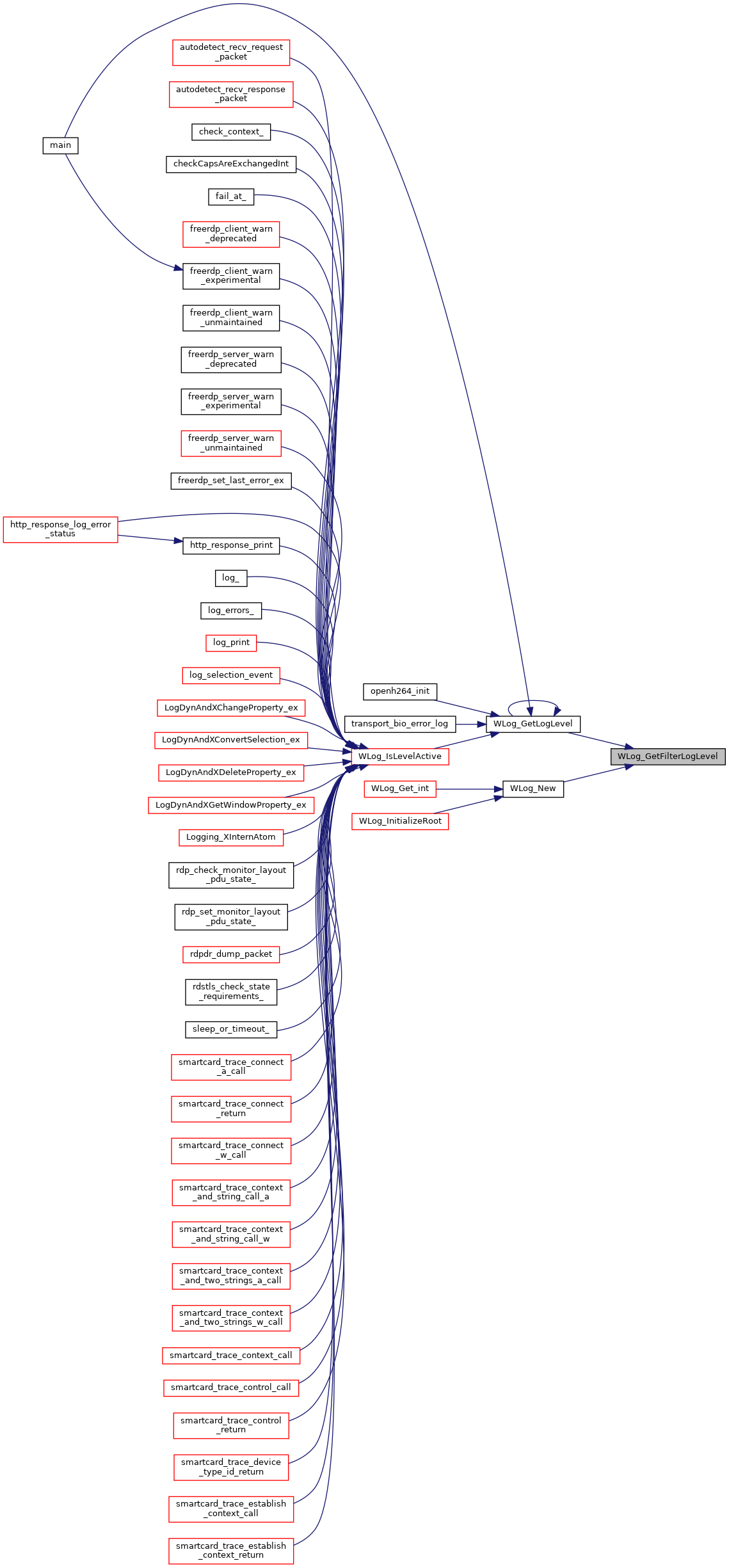## ◆ WLog_GetLogLevel()

 DWORD WLog_GetLogLevel ( wLog * log )
Here is the call graph for this function: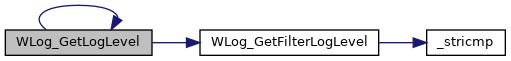Here is the caller graph for this function: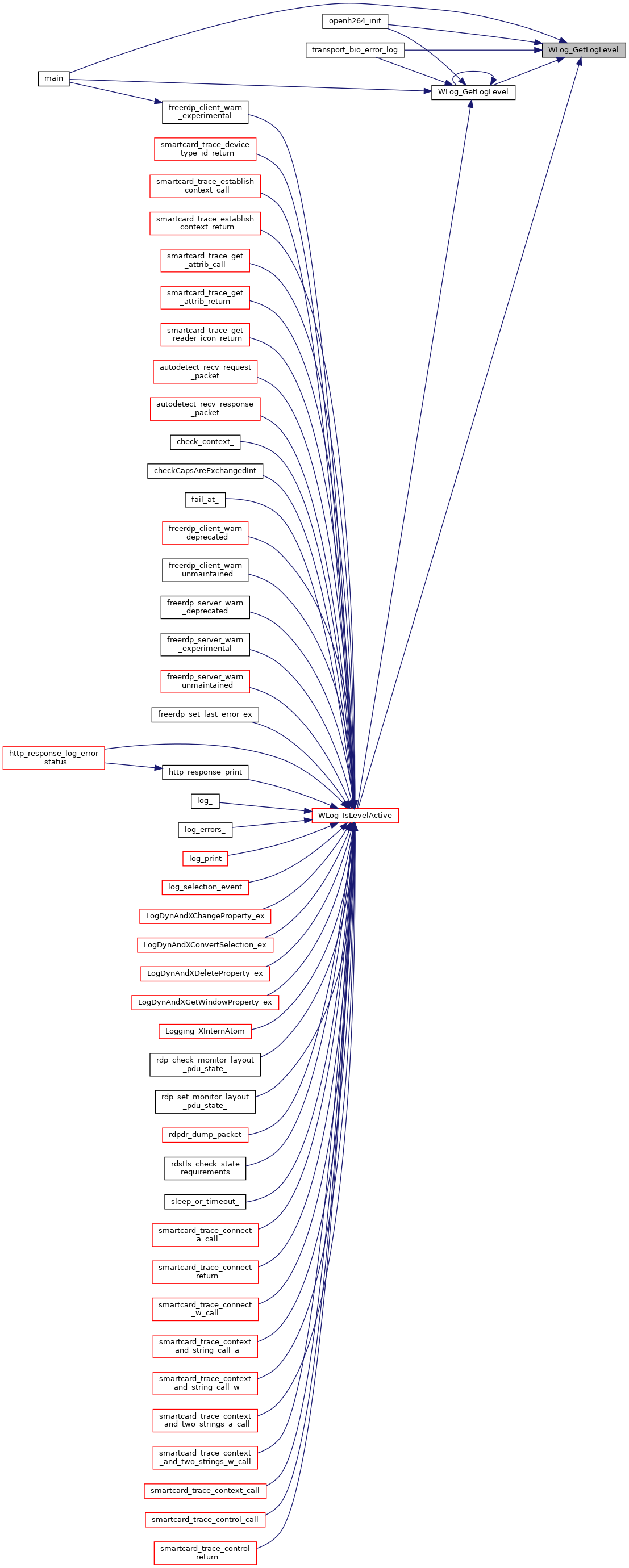## ◆ WLog_GetRoot()

 wLog* WLog_GetRoot ( void )
Here is the call graph for this function: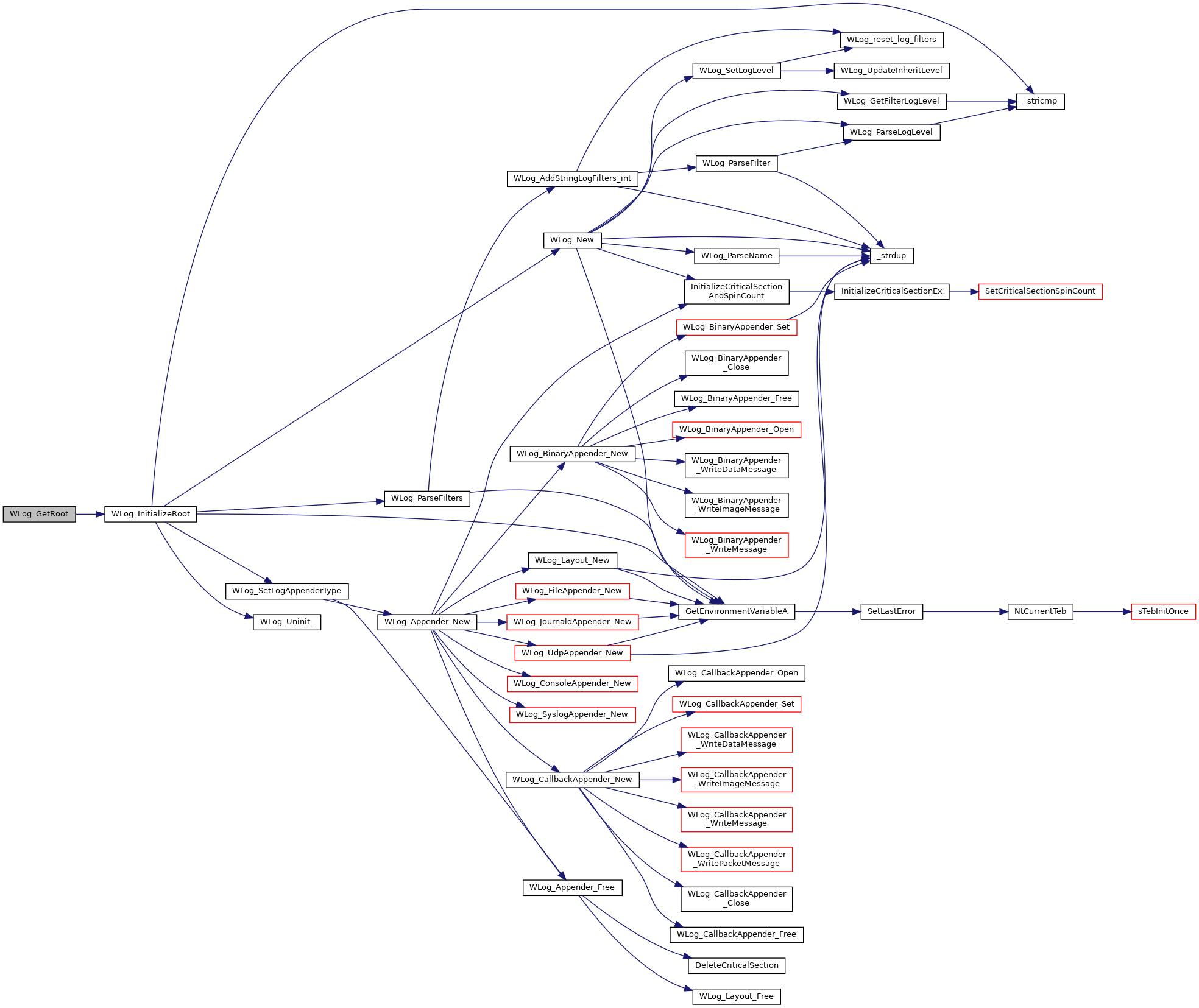Here is the caller graph for this function: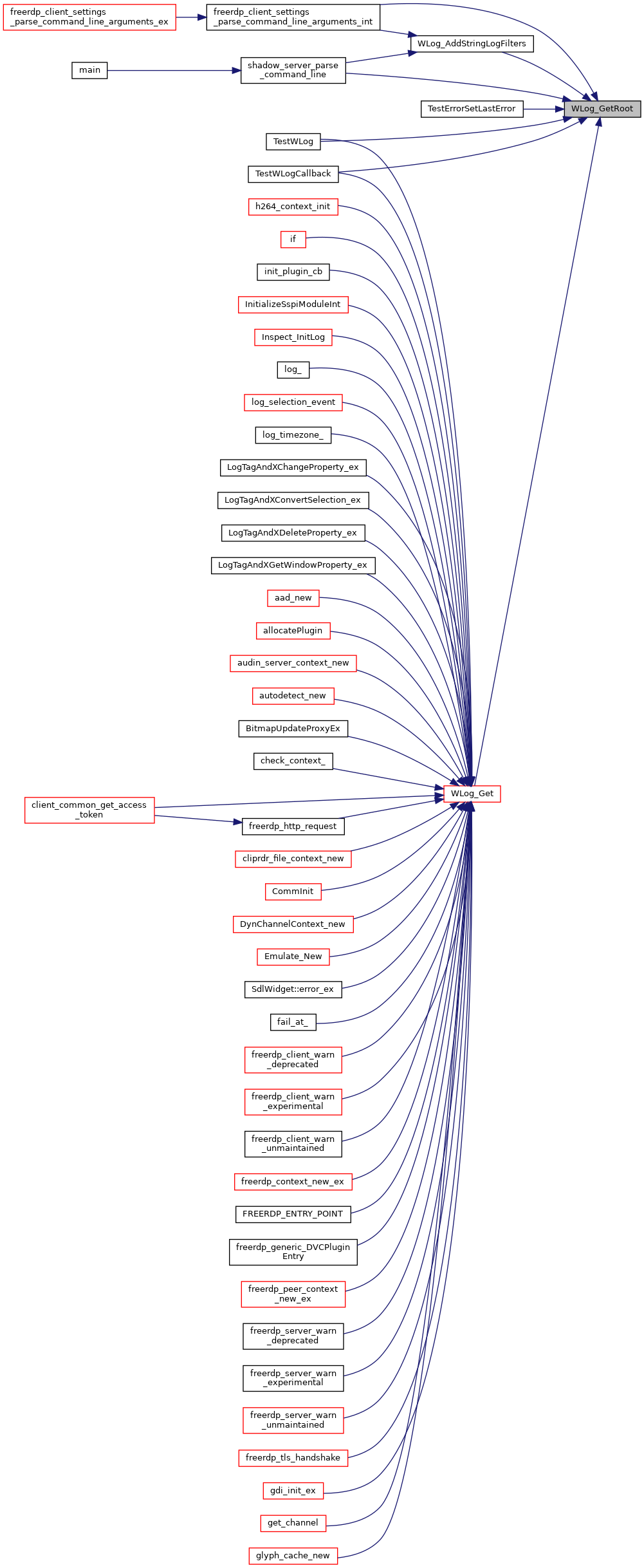## ◆ WLog_InitializeRoot()

 static BOOL CALLBACK WLog_InitializeRoot ( PINIT_ONCE InitOnce, PVOID Parameter, PVOID * Context )
static
Here is the call graph for this function: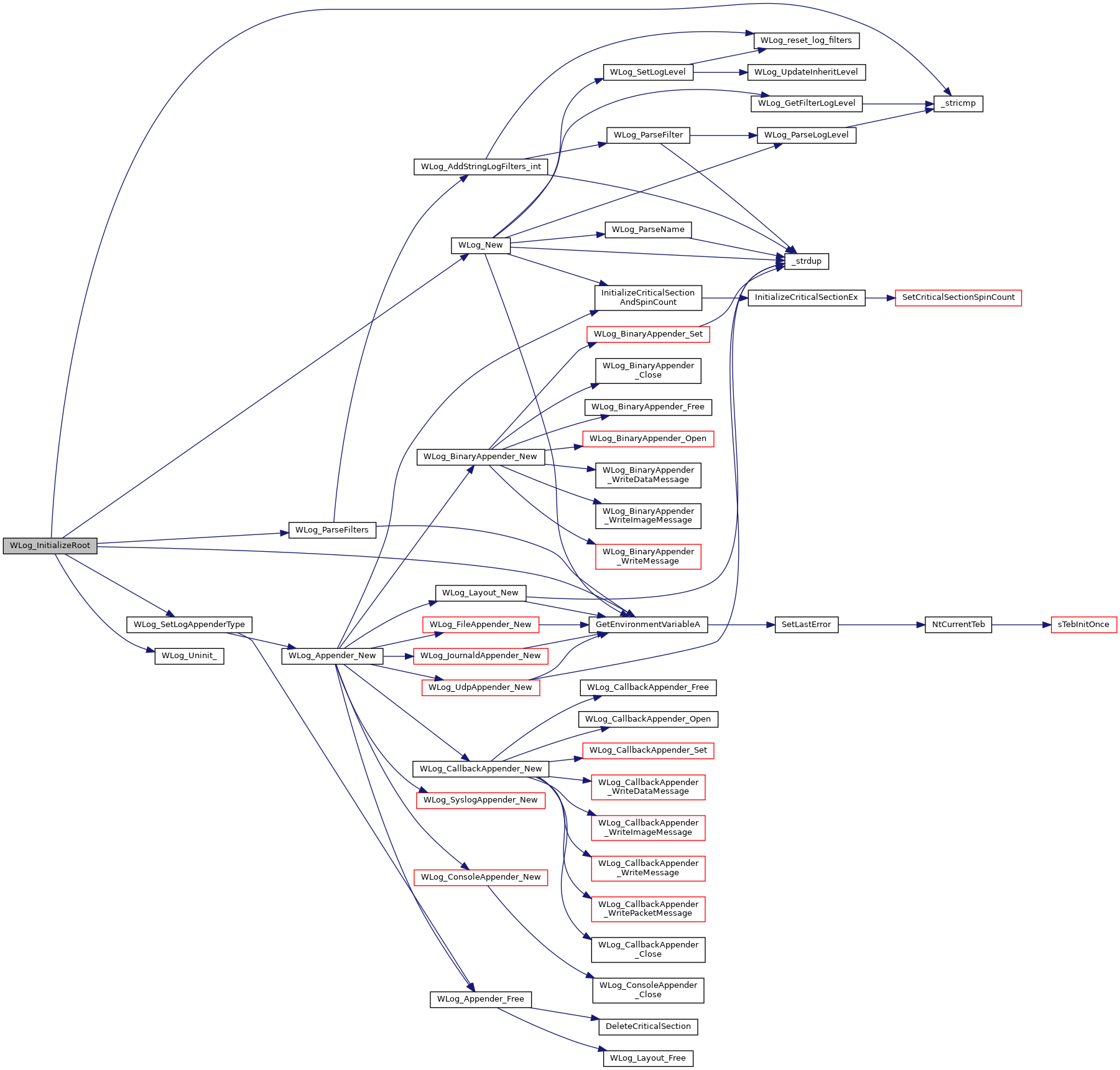Here is the caller graph for this function: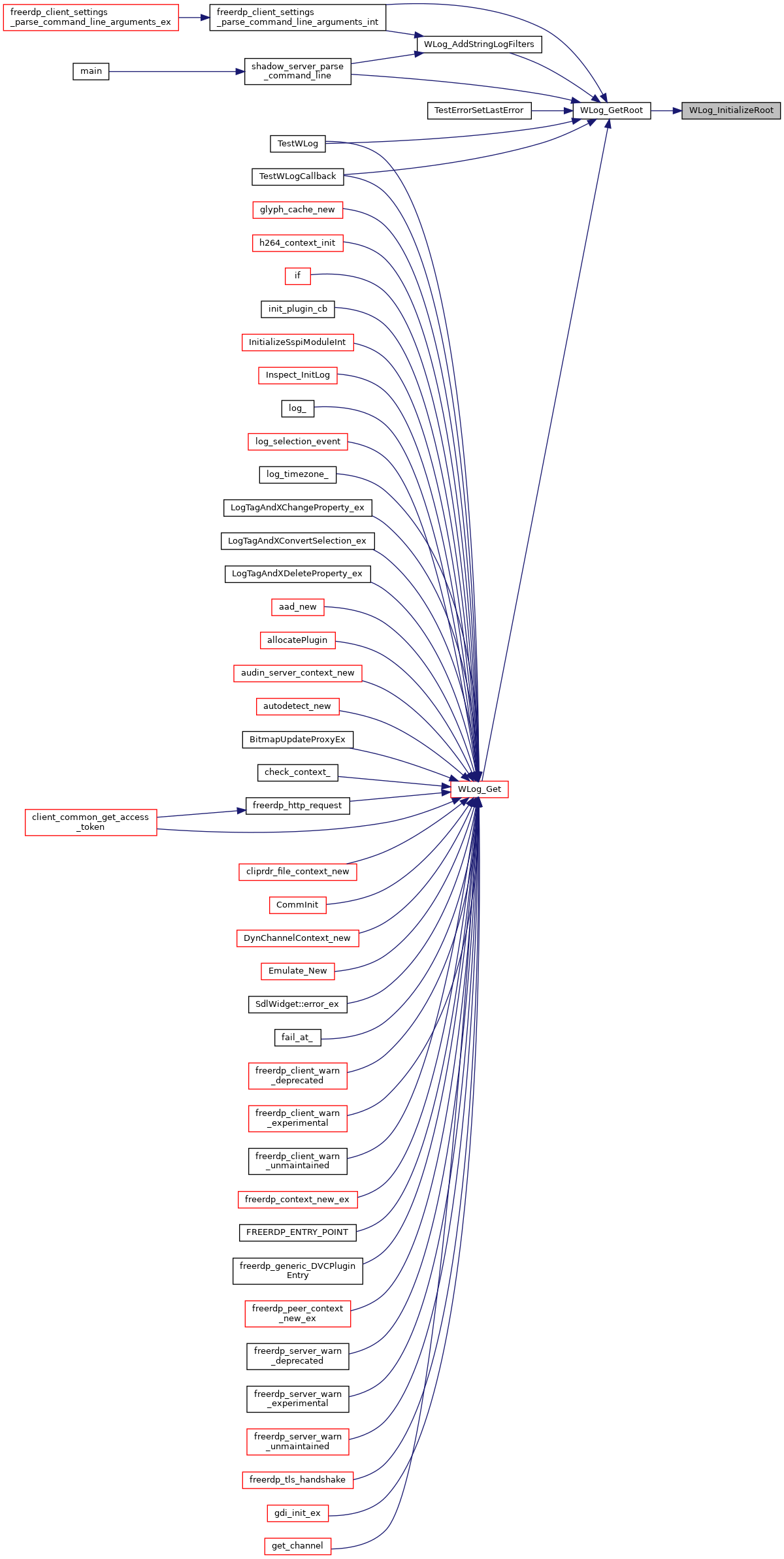## ◆ WLog_IsLevelActive()

 BOOL WLog_IsLevelActive ( wLog * _log, DWORD _log_level )
Here is the call graph for this function: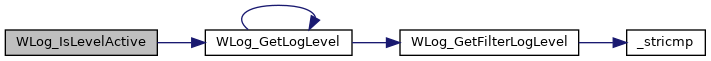## ◆ WLog_Lock()

 static void WLog_Lock ( wLog * log )
static
Here is the call graph for this function: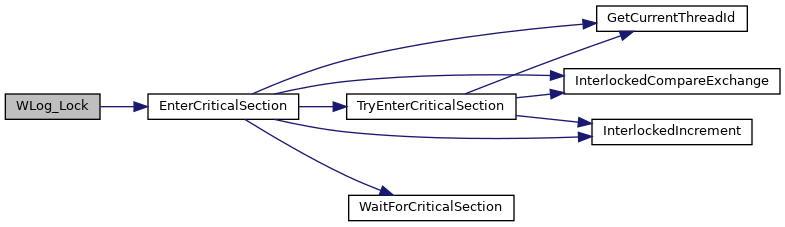Here is the caller graph for this function: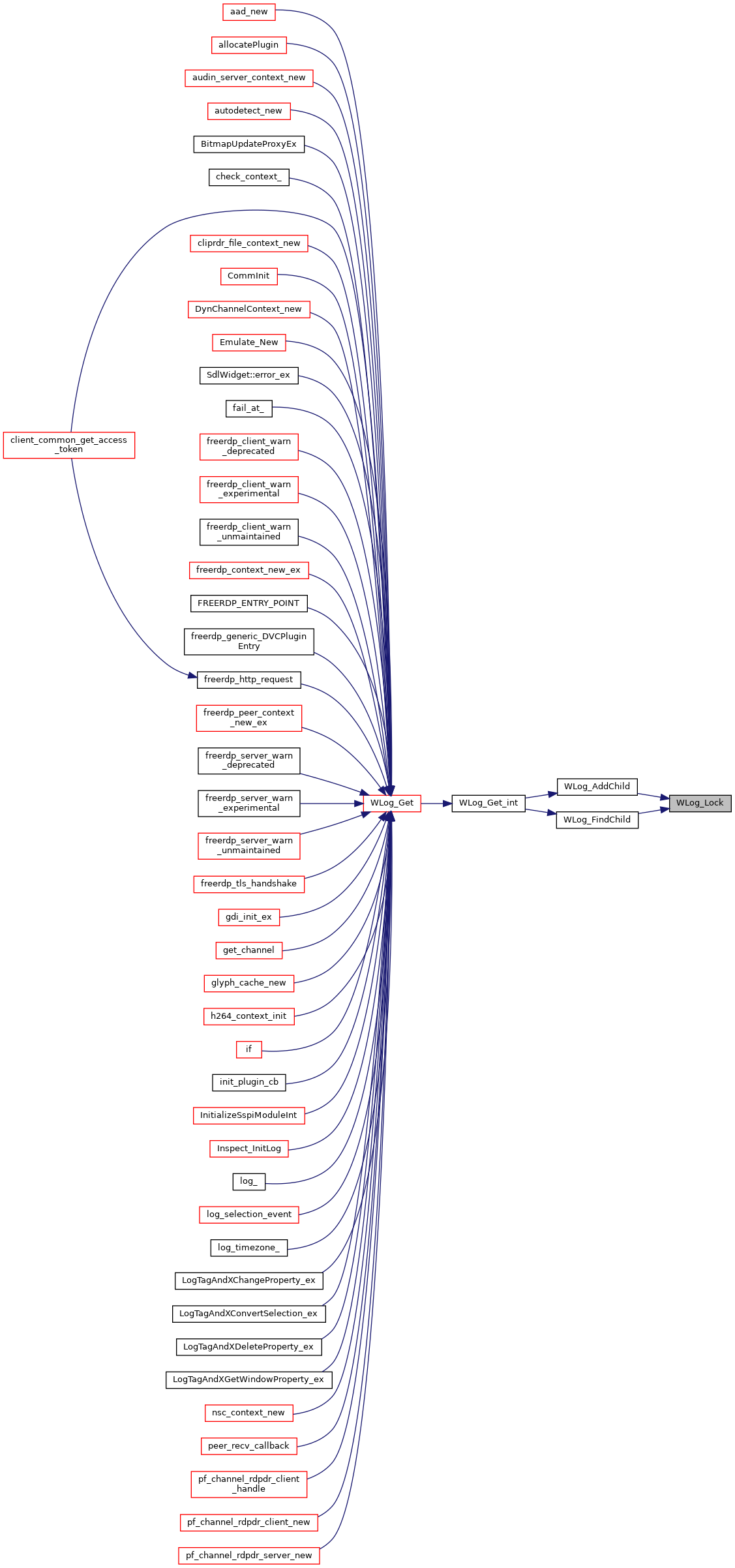## ◆ WLog_New()

 wLog * WLog_New ( LPCSTR name, wLog * rootLogger )
static
Here is the call graph for this function:Here is the caller graph for this function: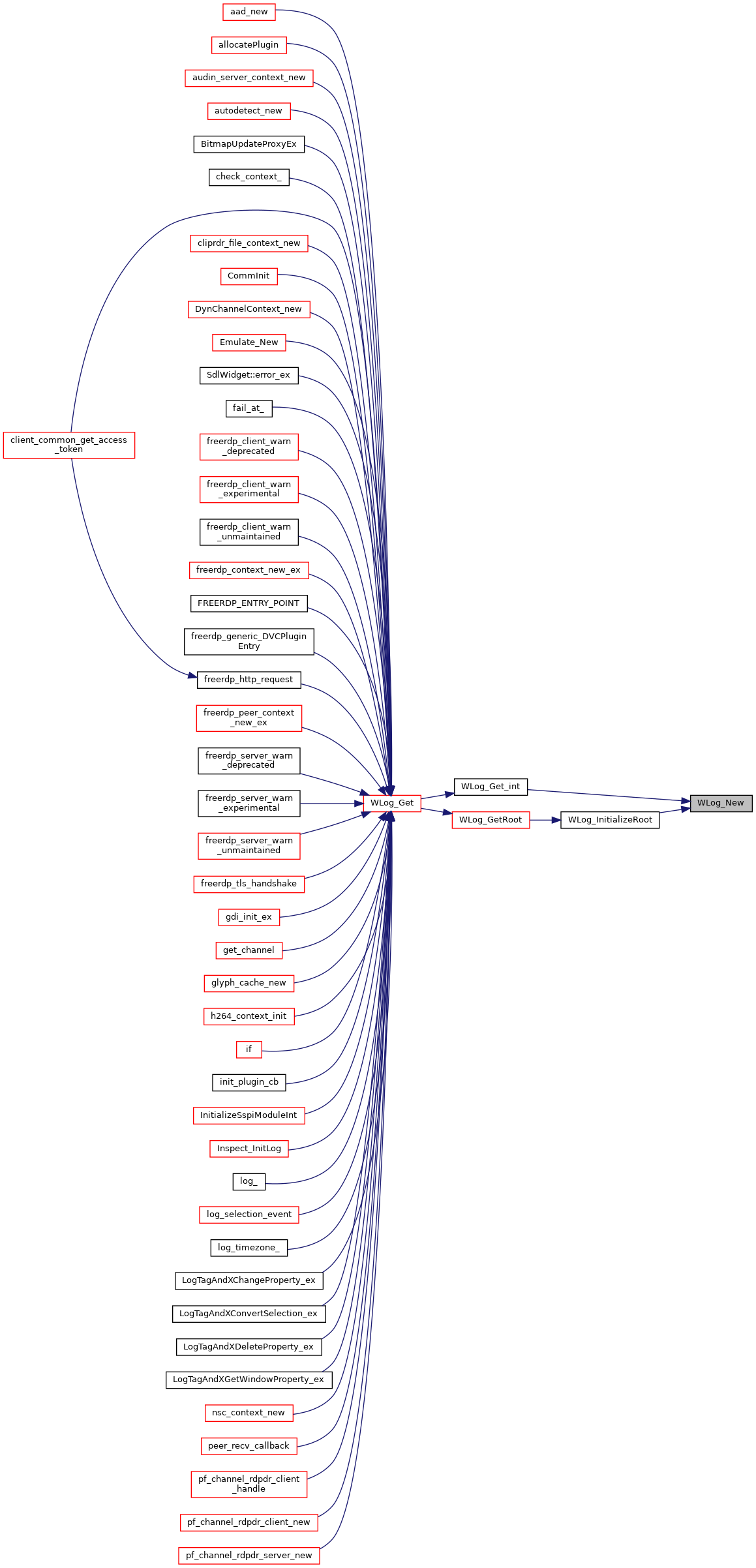## ◆ WLog_ParseFilter()

 BOOL WLog_ParseFilter ( wLog * root, wLogFilter * filter, LPCSTR name )
static
Here is the call graph for this function: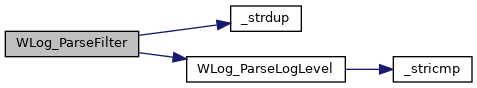Here is the caller graph for this function: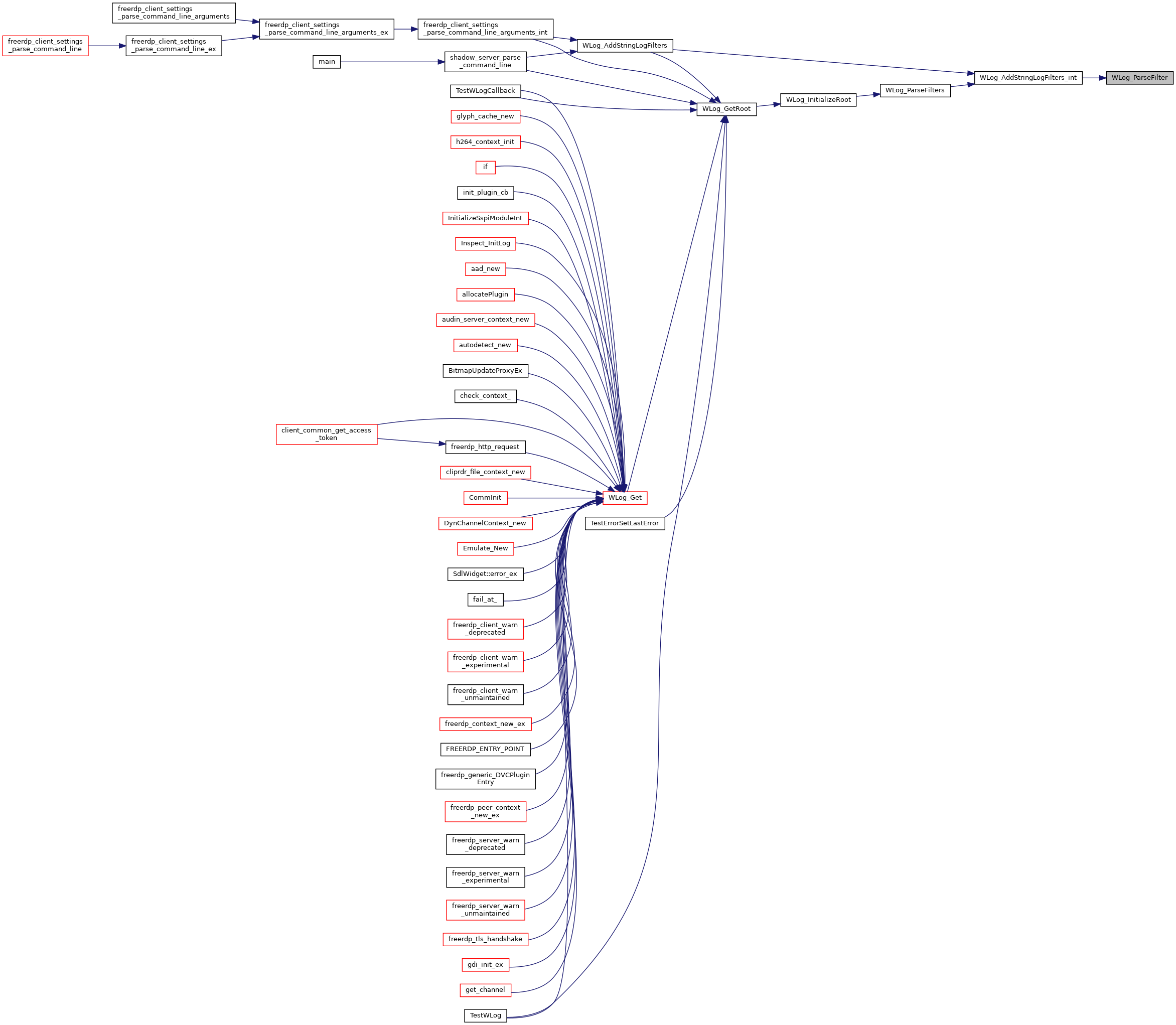## ◆ WLog_ParseFilters()

 BOOL WLog_ParseFilters ( wLog * root )
static
Here is the call graph for this function: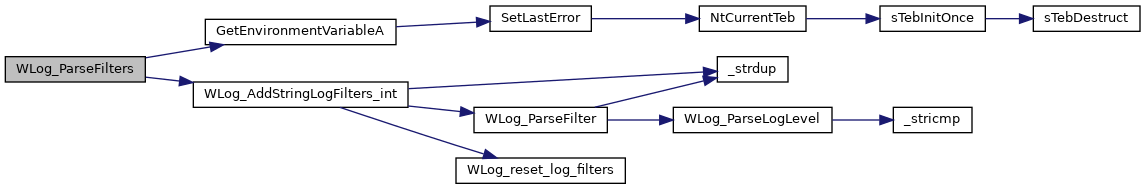Here is the caller graph for this function: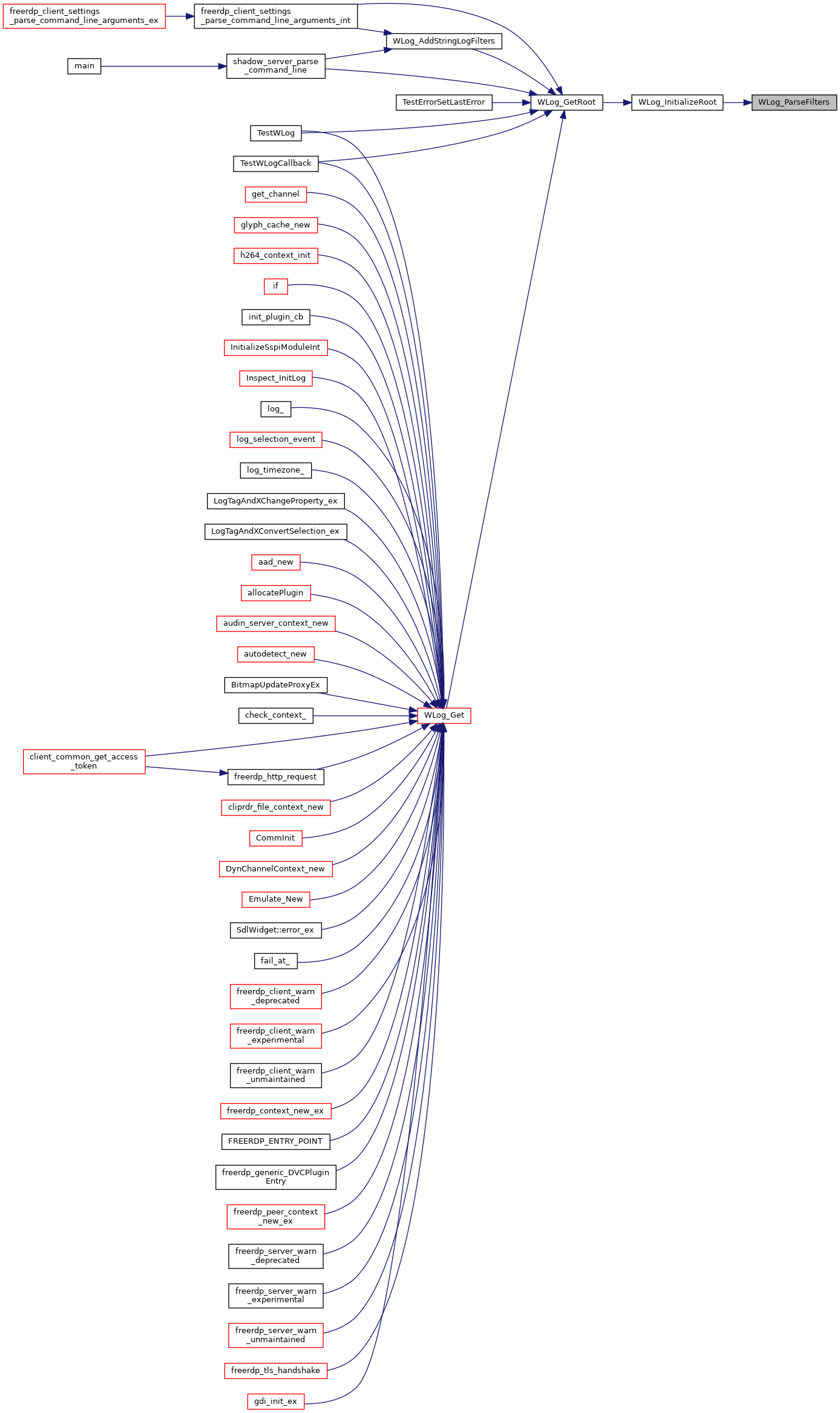## ◆ WLog_ParseLogLevel()

 int WLog_ParseLogLevel ( LPCSTR level )
static
Here is the call graph for this function: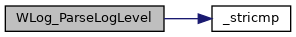Here is the caller graph for this function: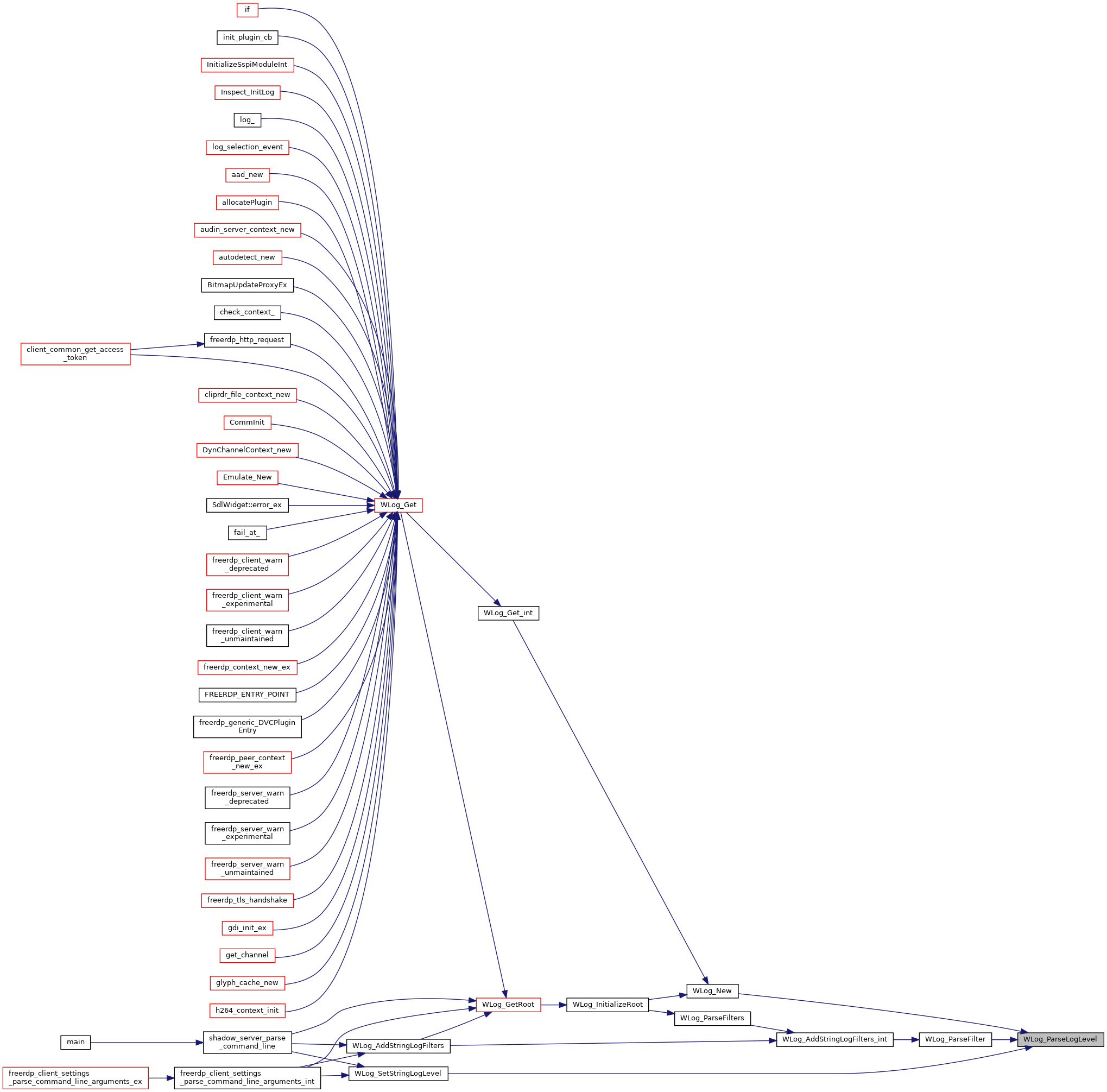## ◆ WLog_ParseName()

 static BOOL WLog_ParseName ( wLog * log, LPCSTR name )
static
Here is the call graph for this function: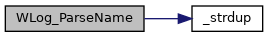Here is the caller graph for this function: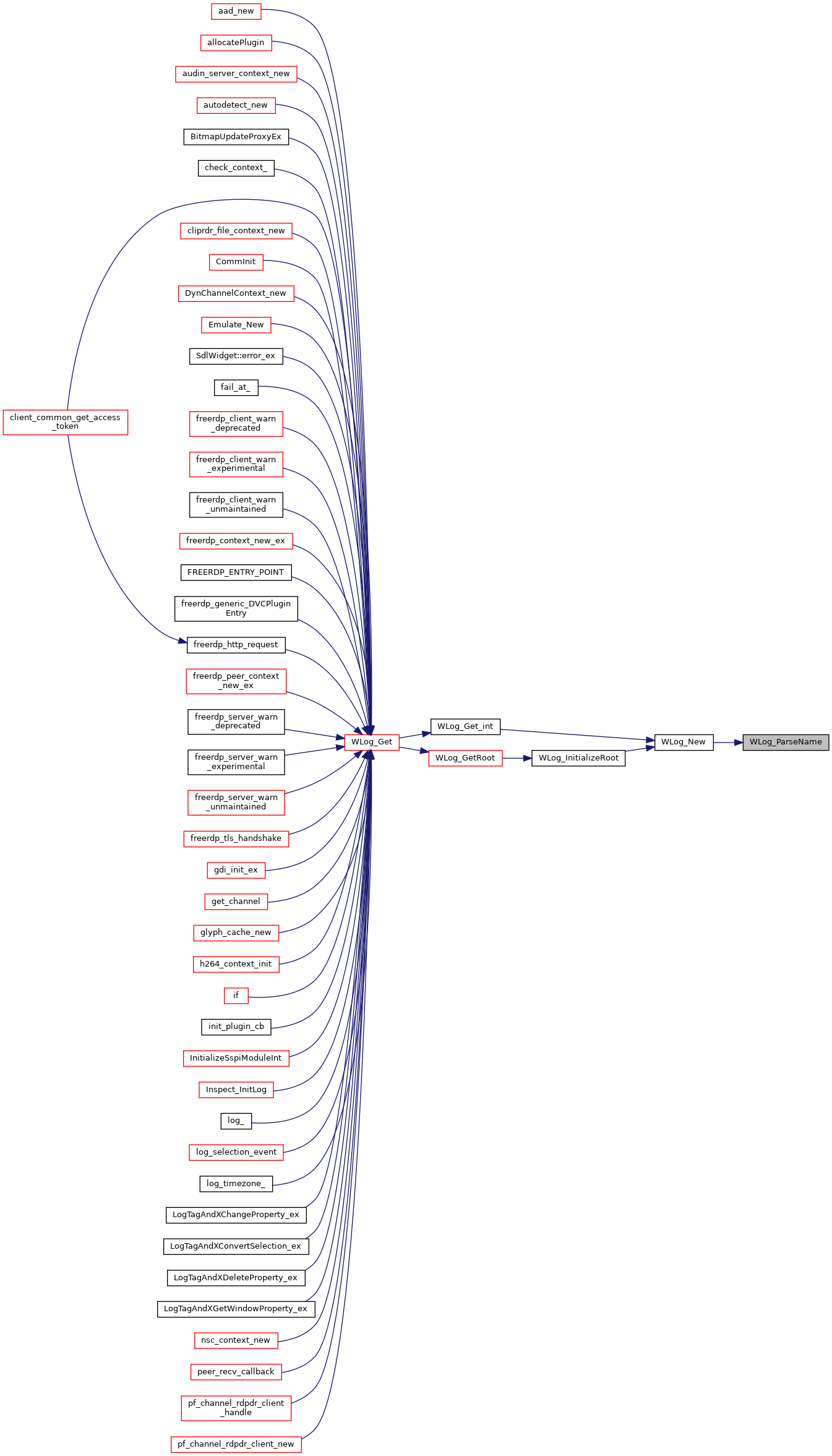## ◆ WLog_PrintMessage()

 BOOL WLog_PrintMessage ( wLog * log, DWORD type, DWORD level, size_t line, const char * file, const char * function, ... )
Here is the call graph for this function: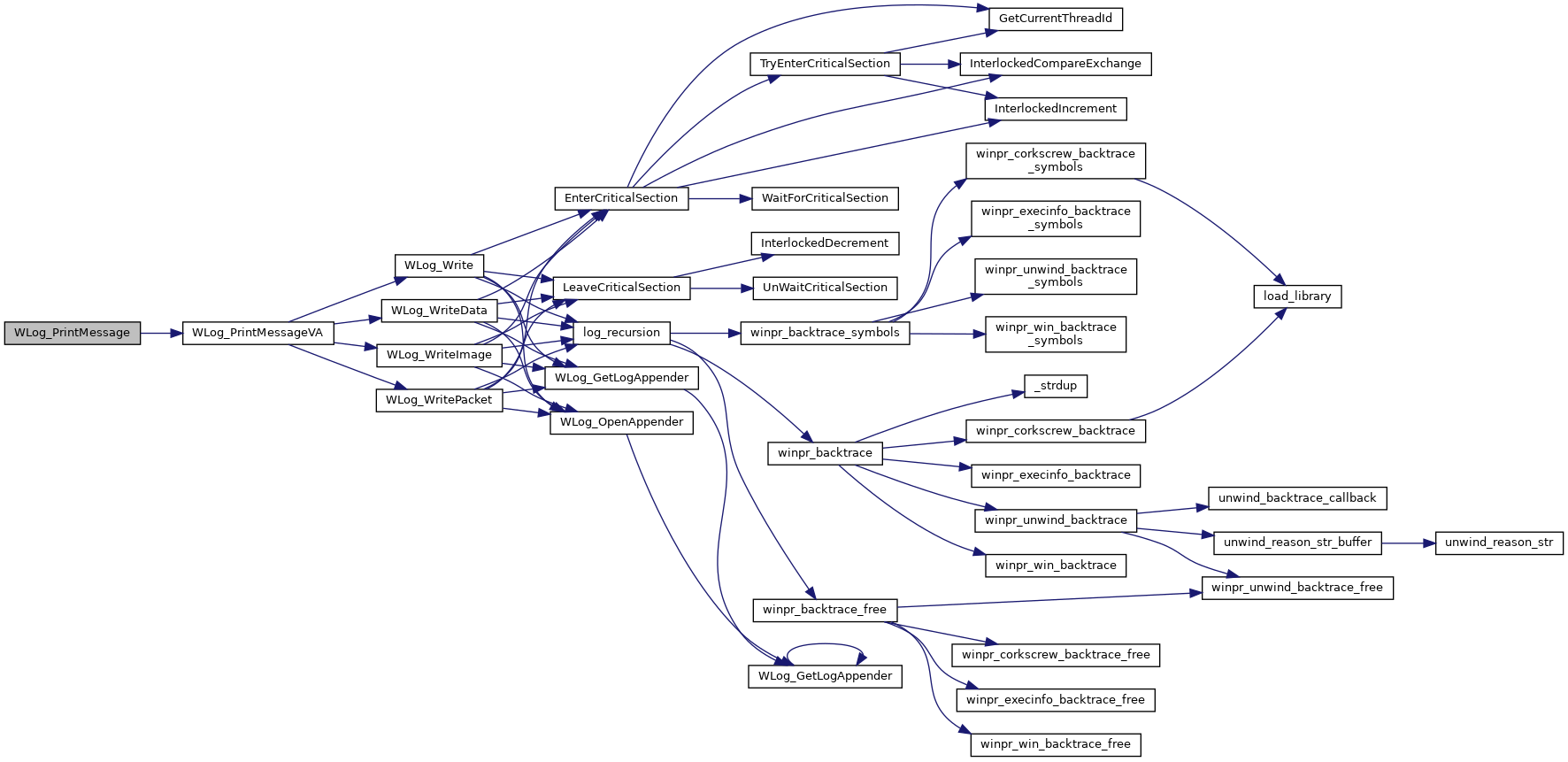Here is the caller graph for this function: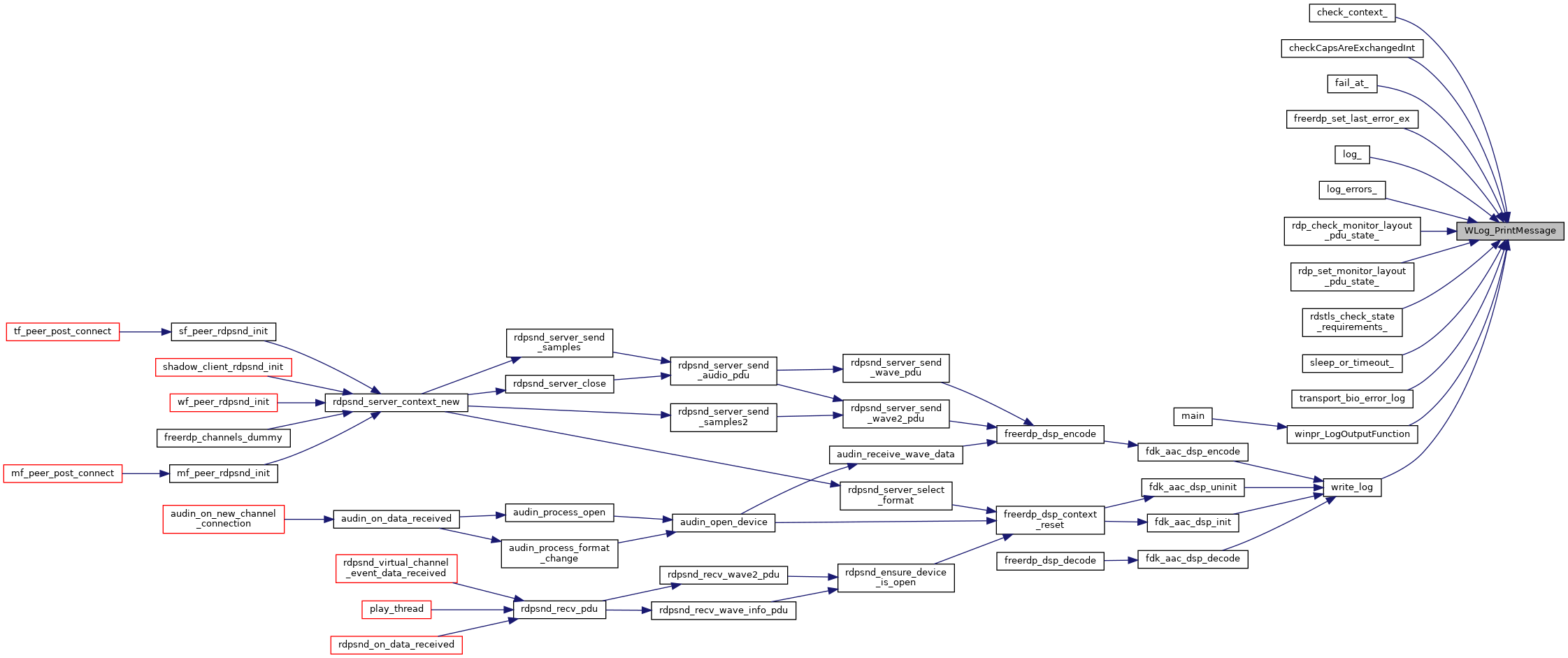## ◆ WLog_PrintMessageVA()

 BOOL WLog_PrintMessageVA ( wLog * log, DWORD type, DWORD level, size_t line, const char * file, const char * function, va_list args )
Here is the call graph for this function: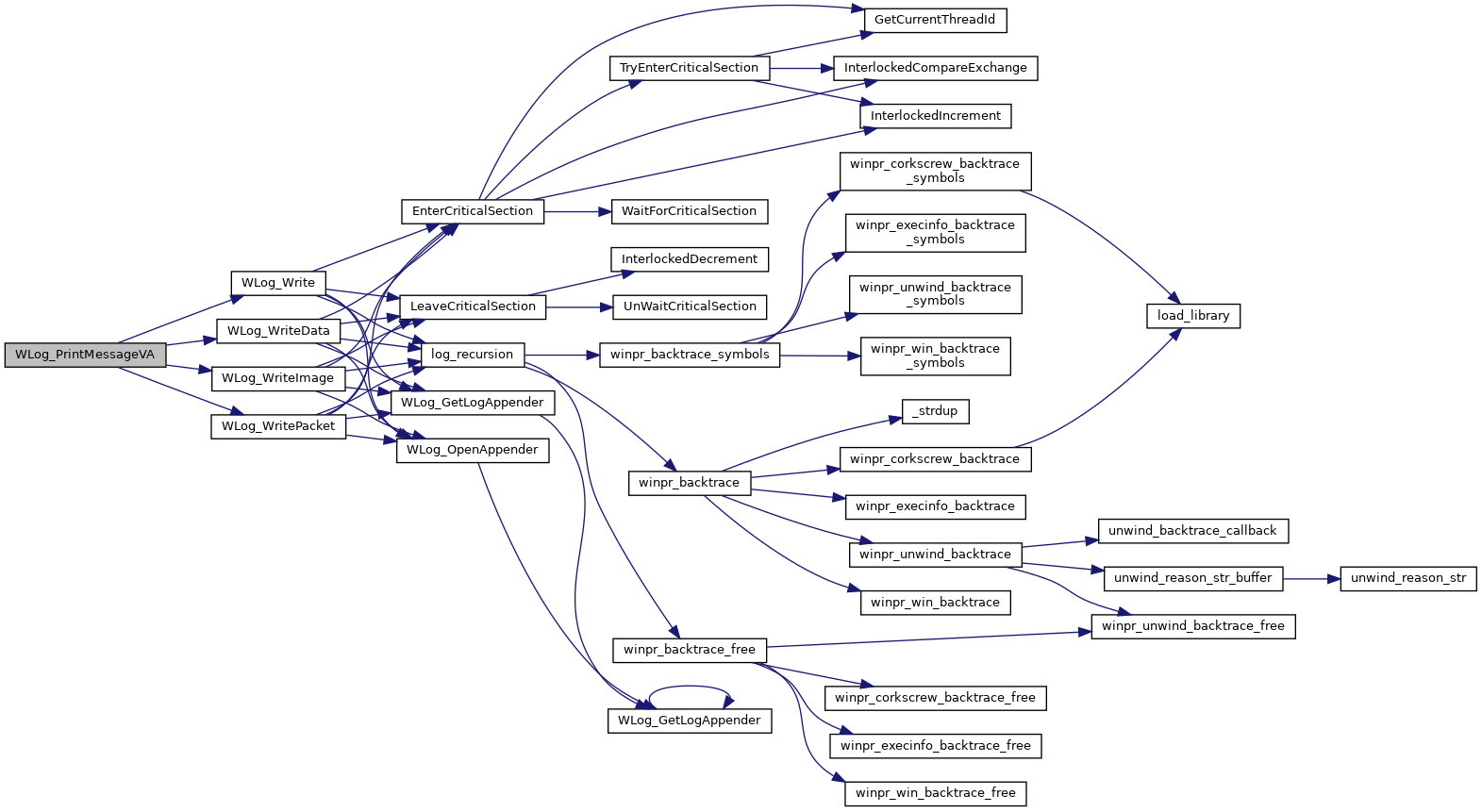Here is the caller graph for this function:## ◆ WLog_reset_log_filters()

 static BOOL WLog_reset_log_filters ( wLog * log )
static
Here is the caller graph for this function: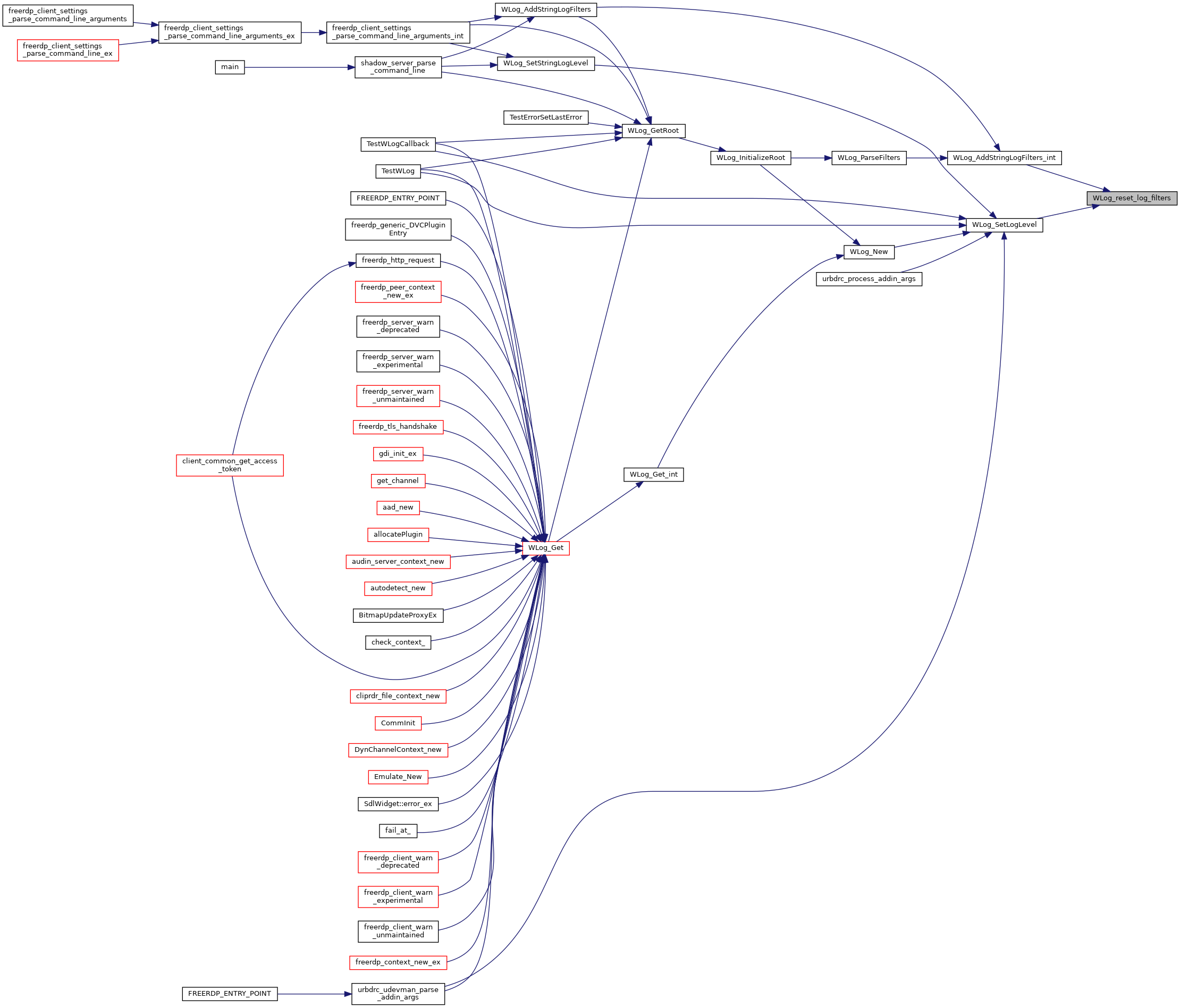## ◆ WLog_SetContext()

 BOOL WLog_SetContext ( wLog * log, const char *(*)(void *) fkt, void * context )

Set a custom context for a dynamic logger. This can be used to print a customized prefix, e.g. some session id for a specific context.

Parameters
 log The logger to ste the context for. Must not be NULL fkt A function pointer that is called to get the custimized string. context A context fkt is called with. Caller must ensure it is still allocated when log is used
Returns
TRUE for success, FALSE otherwise.
Here is the caller graph for this function: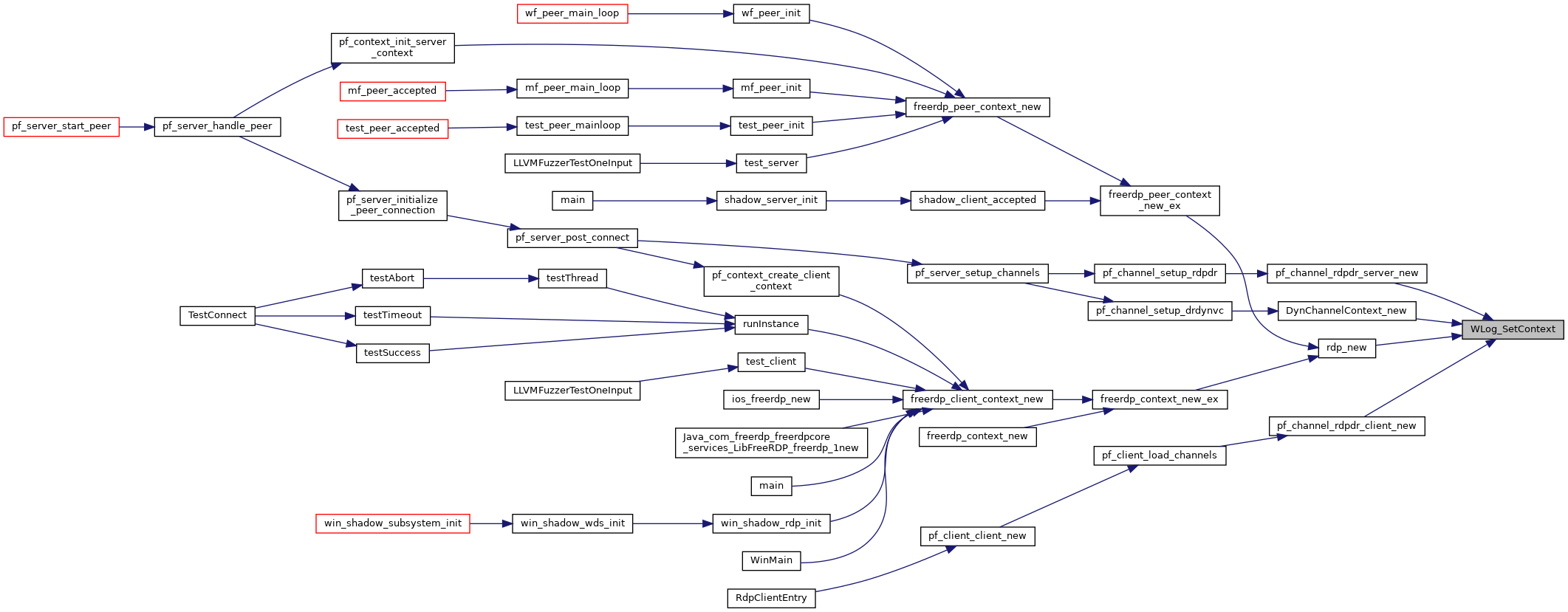## ◆ WLog_SetLogLevel()

 BOOL WLog_SetLogLevel ( wLog * log, DWORD logLevel )
Here is the call graph for this function: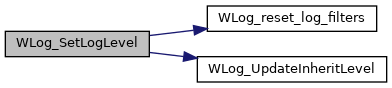Here is the caller graph for this function: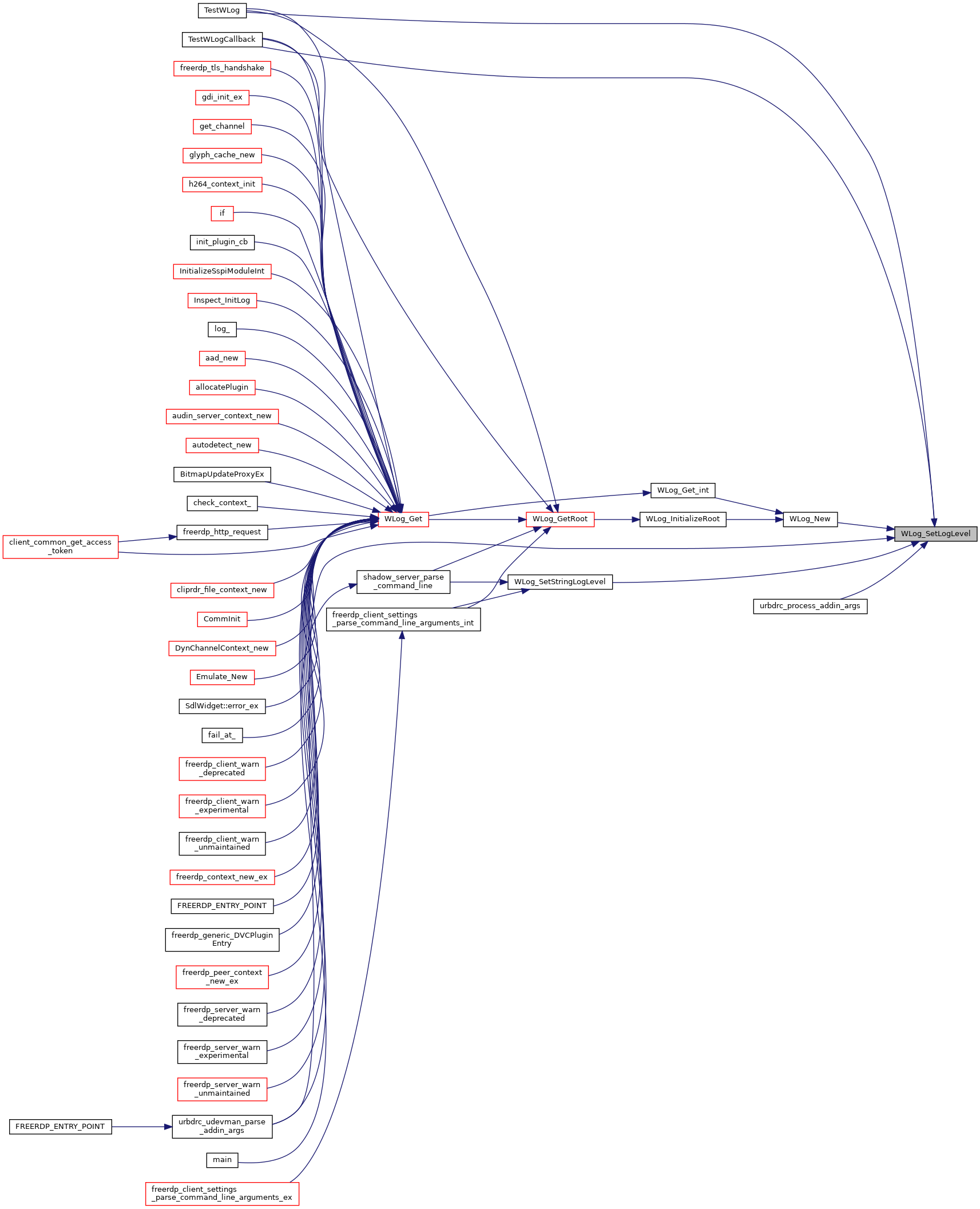## ◆ WLog_SetStringLogLevel()

 BOOL WLog_SetStringLogLevel ( wLog * log, LPCSTR level )
Here is the call graph for this function: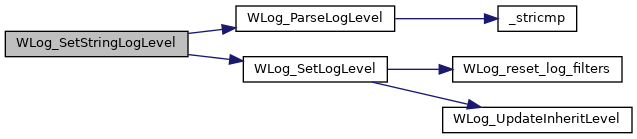Here is the caller graph for this function:## ◆ WLog_Uninit_()

 static void WLog_Uninit_ ( void )
static
Here is the caller graph for this function: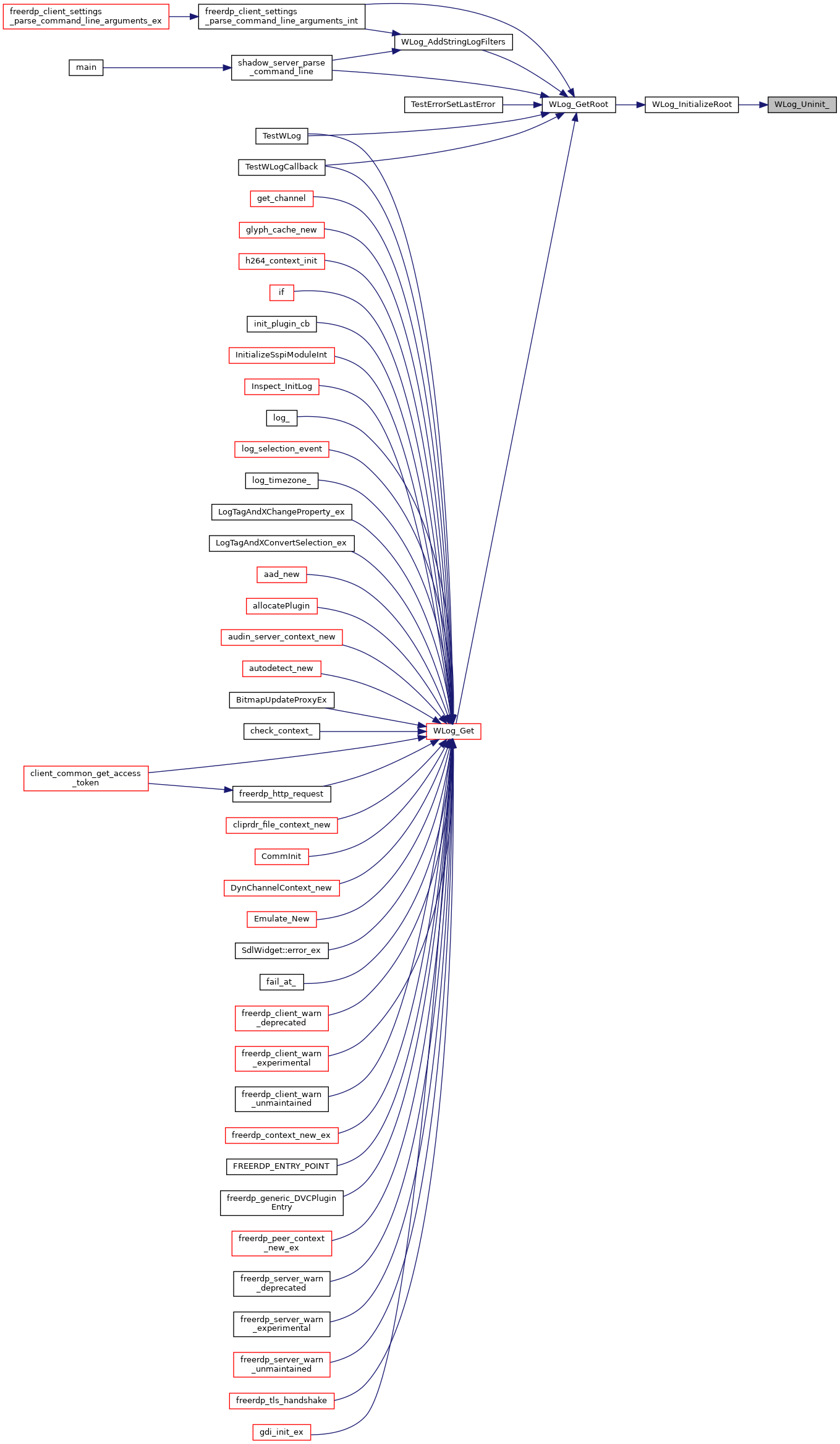## ◆ WLog_Unlock()

 static void WLog_Unlock ( wLog * log )
static
Here is the call graph for this function: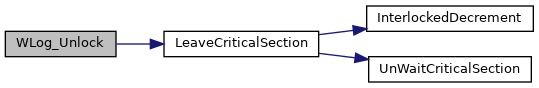Here is the caller graph for this function:## ◆ WLog_UpdateInheritLevel()

 static BOOL WLog_UpdateInheritLevel ( wLog * log, DWORD logLevel )
static
Here is the caller graph for this function: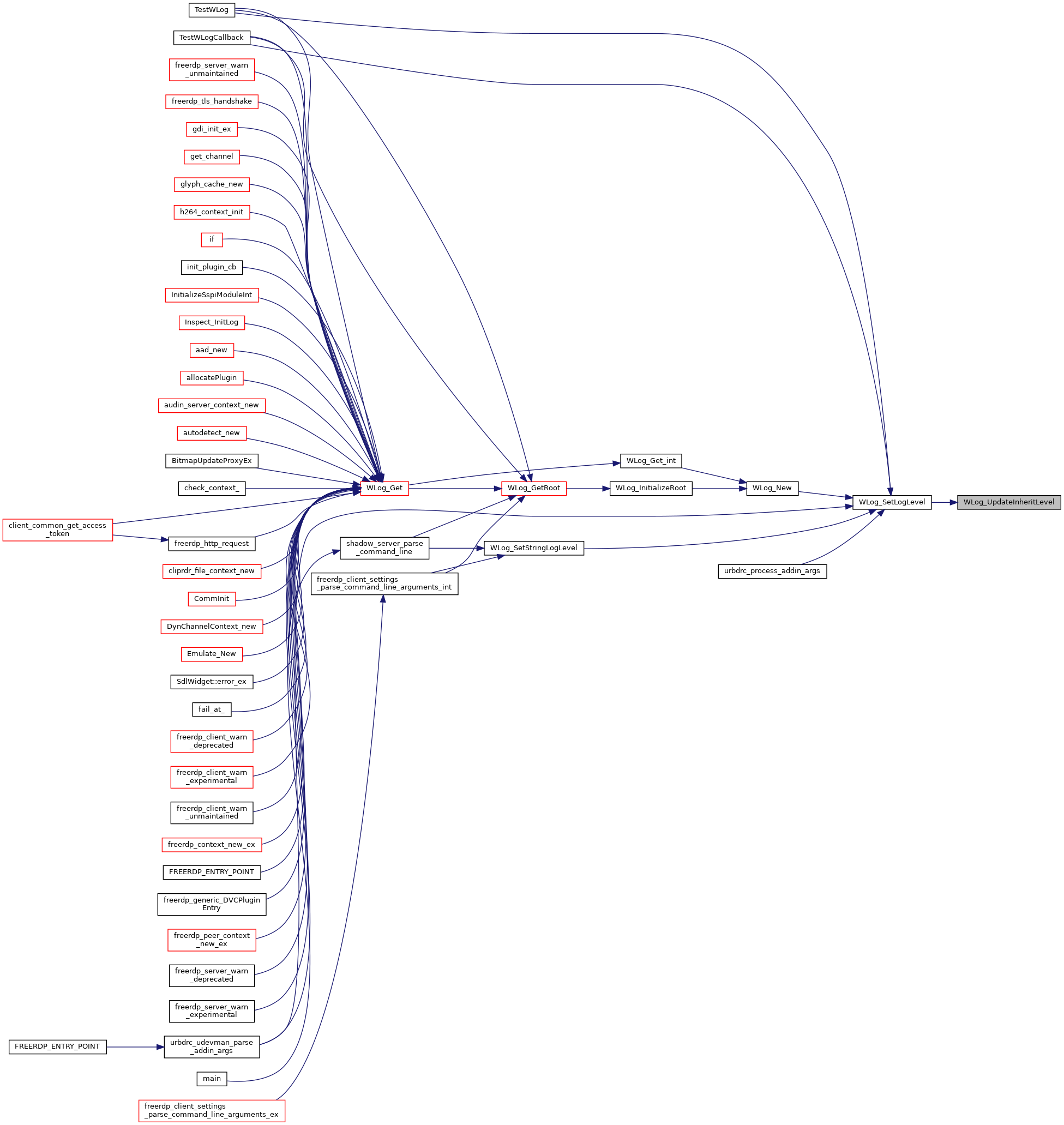## ◆ WLog_Write()

 static BOOL WLog_Write ( wLog * log, wLogMessage * message )
static
Here is the call graph for this function: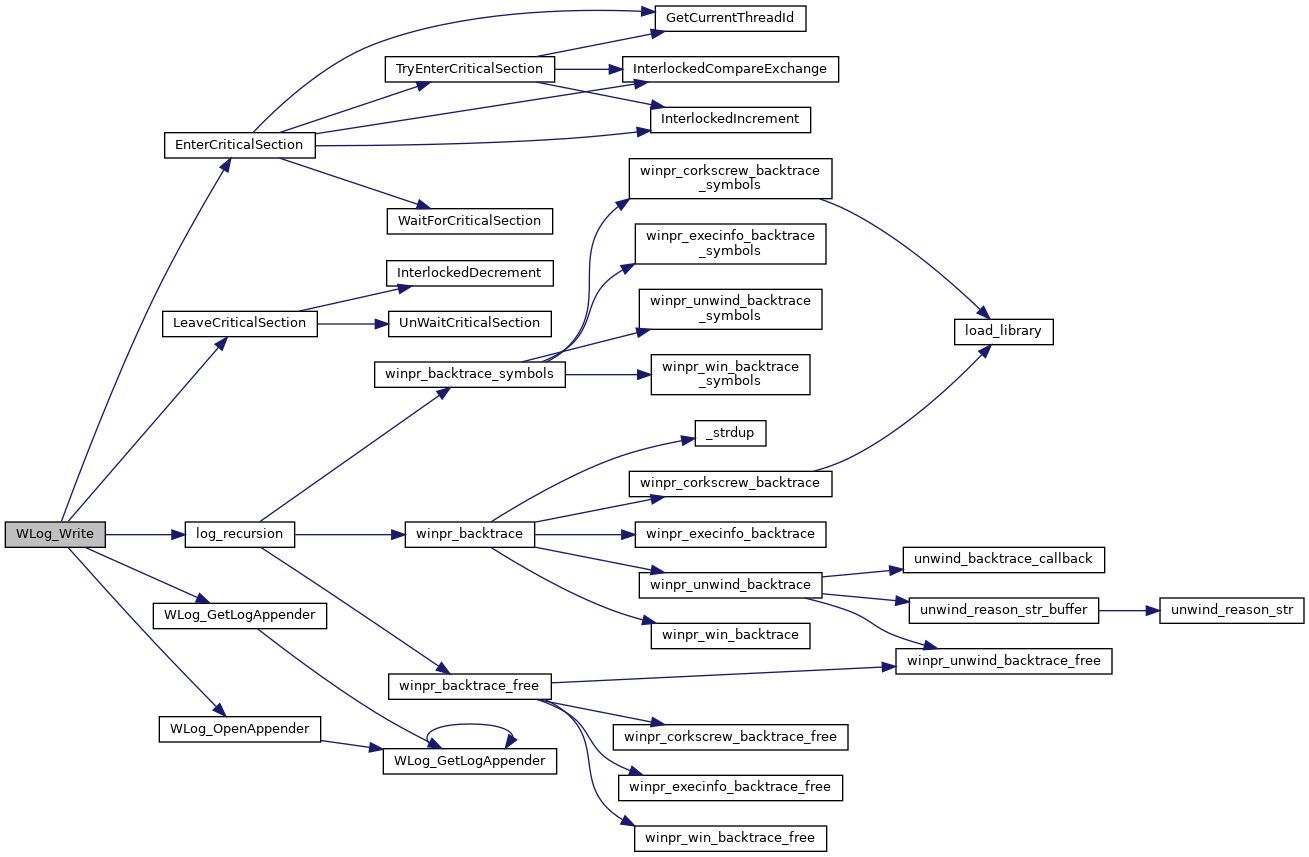Here is the caller graph for this function: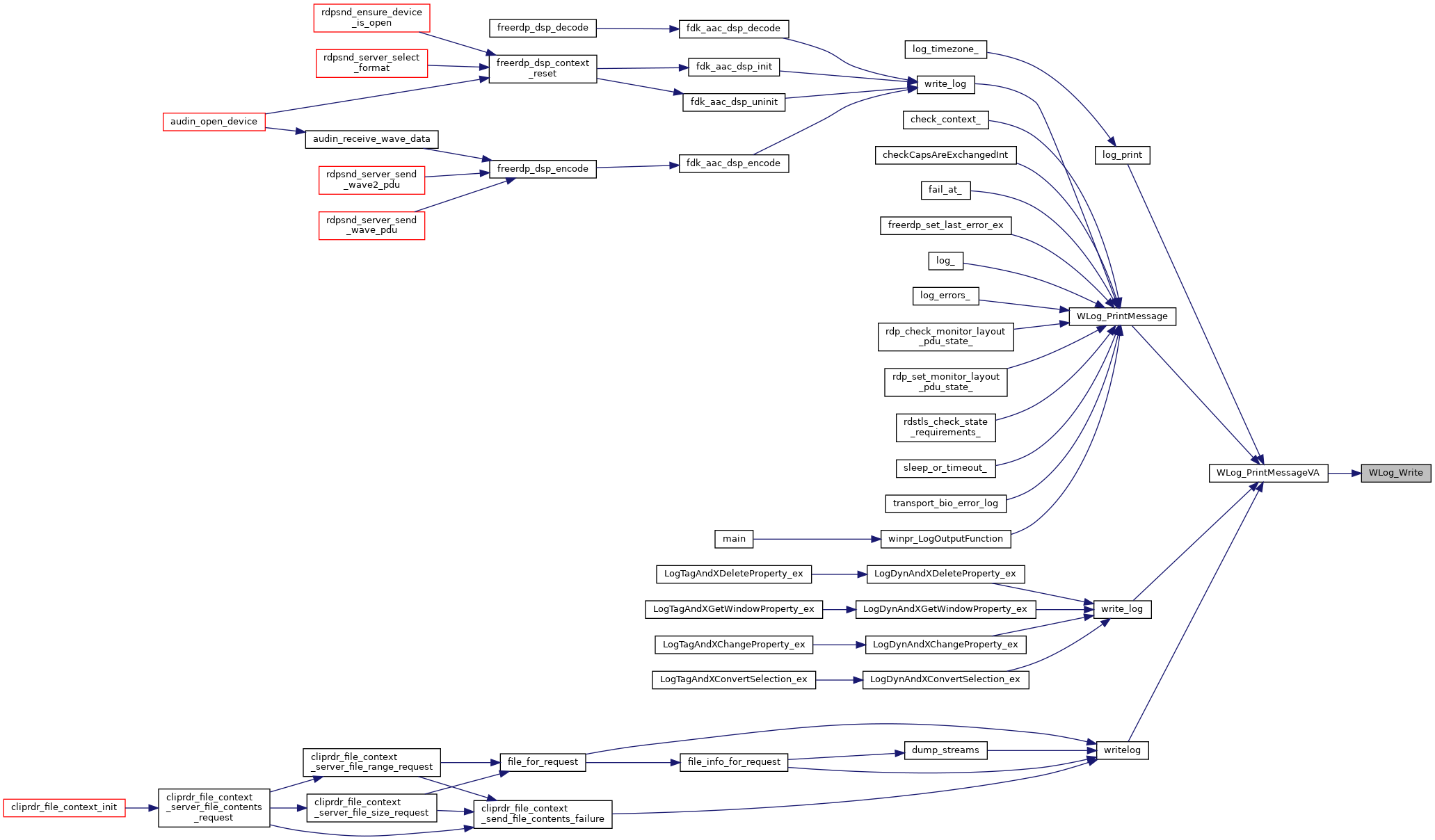## ◆ WLog_WriteData()

 static BOOL WLog_WriteData ( wLog * log, wLogMessage * message )
static
Here is the call graph for this function: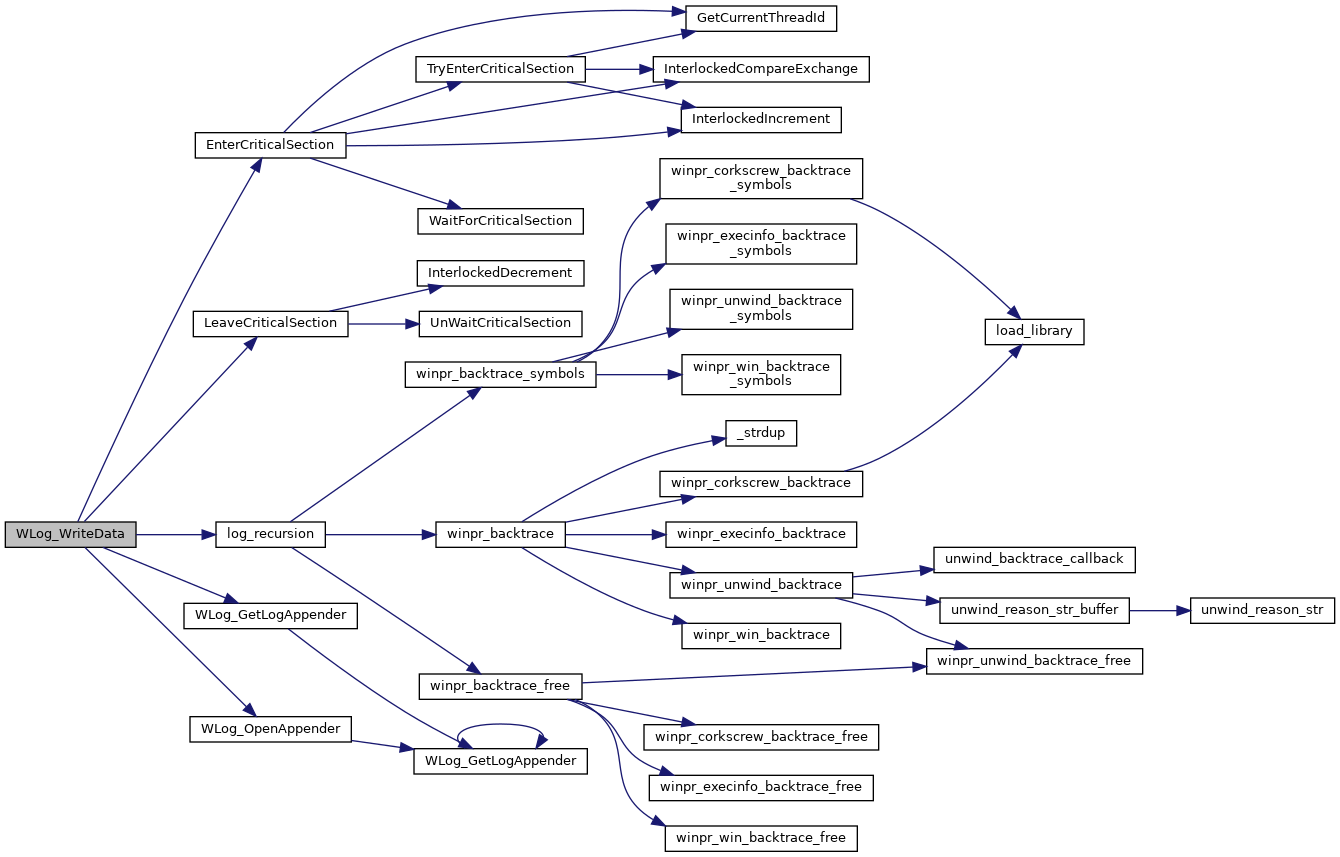Here is the caller graph for this function: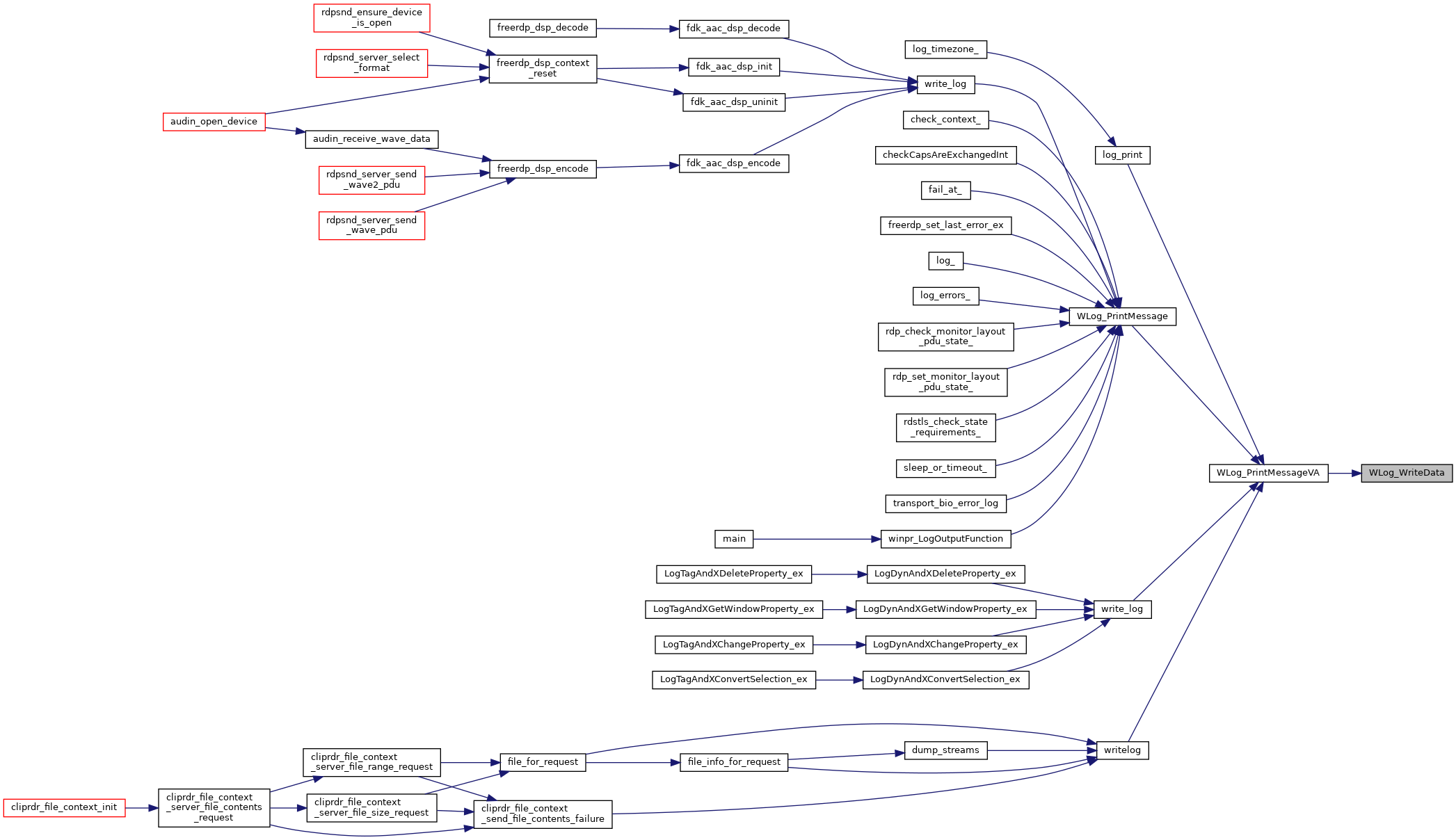## ◆ WLog_WriteImage()

 static BOOL WLog_WriteImage ( wLog * log, wLogMessage * message )
static
Here is the call graph for this function: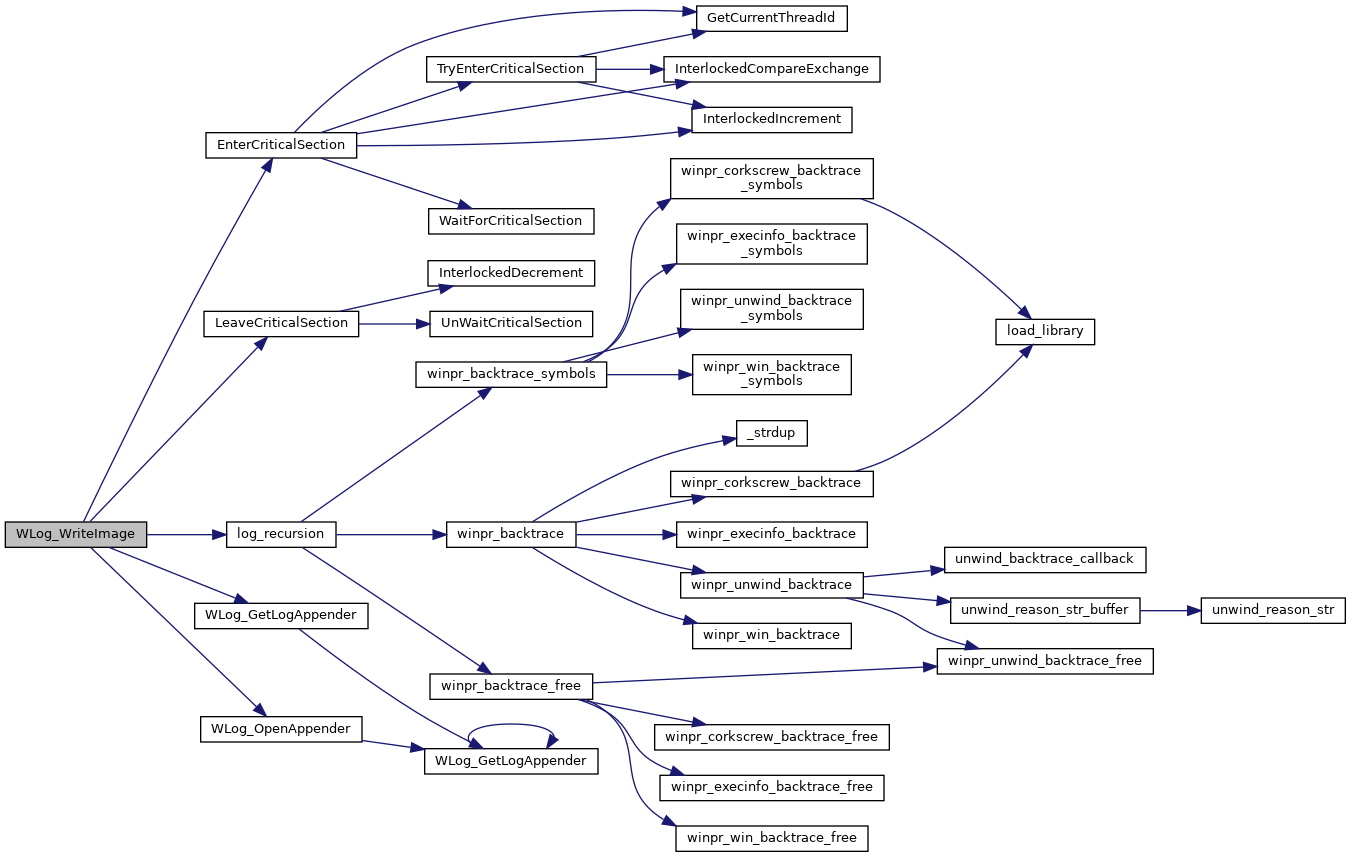Here is the caller graph for this function: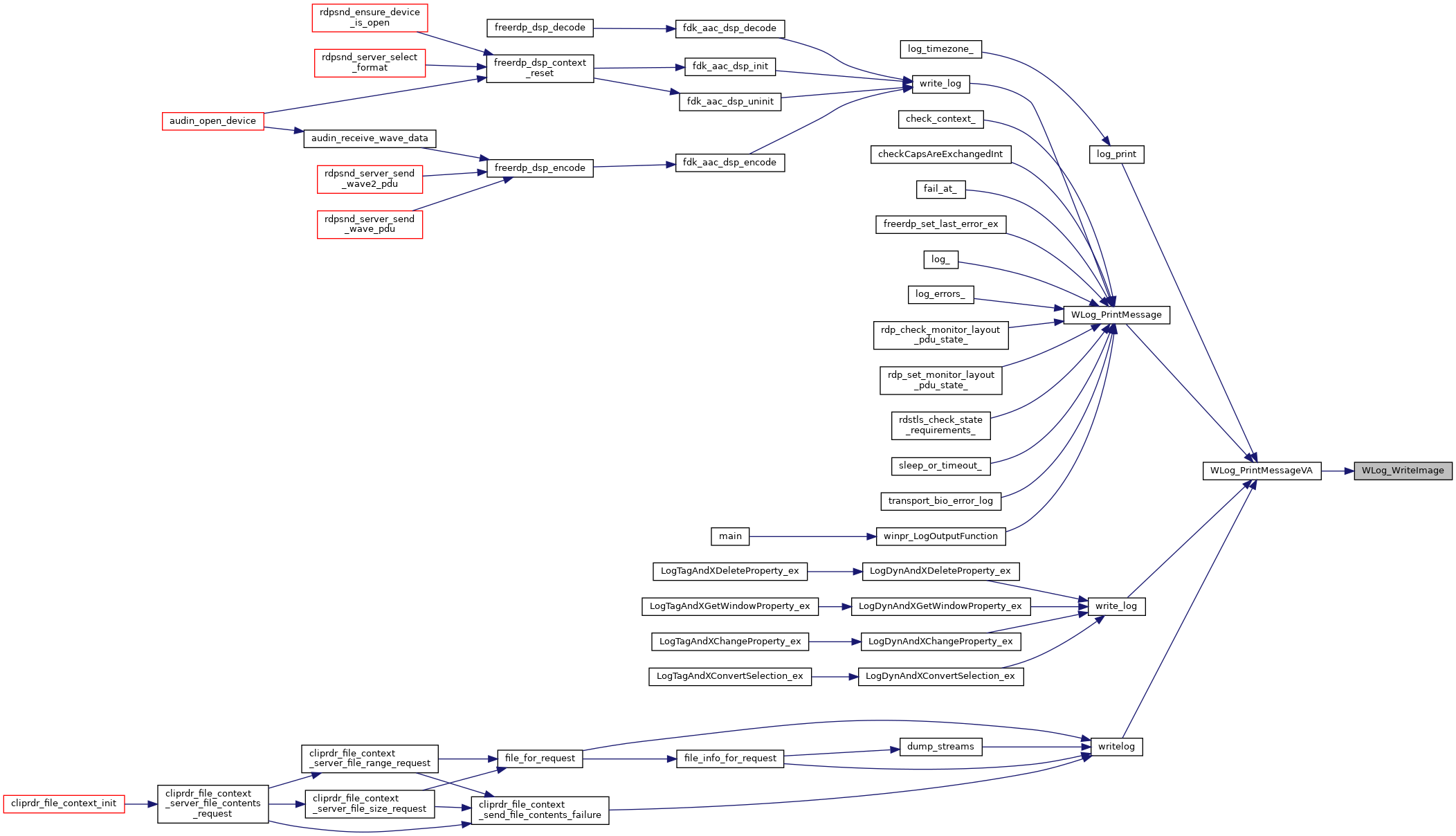## ◆ WLog_WritePacket()

 static BOOL WLog_WritePacket ( wLog * log, wLogMessage * message )
static
Here is the call graph for this function: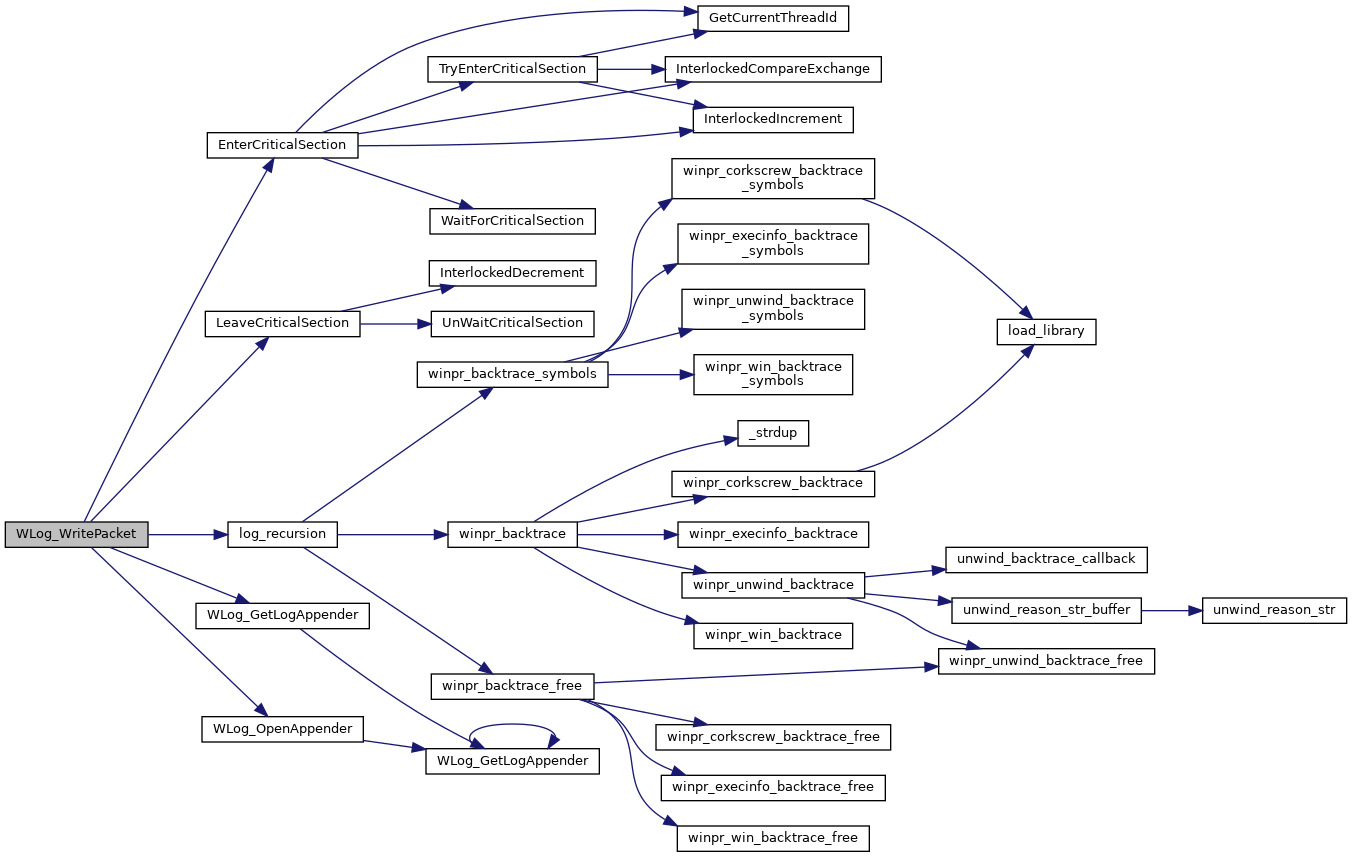Here is the caller graph for this function: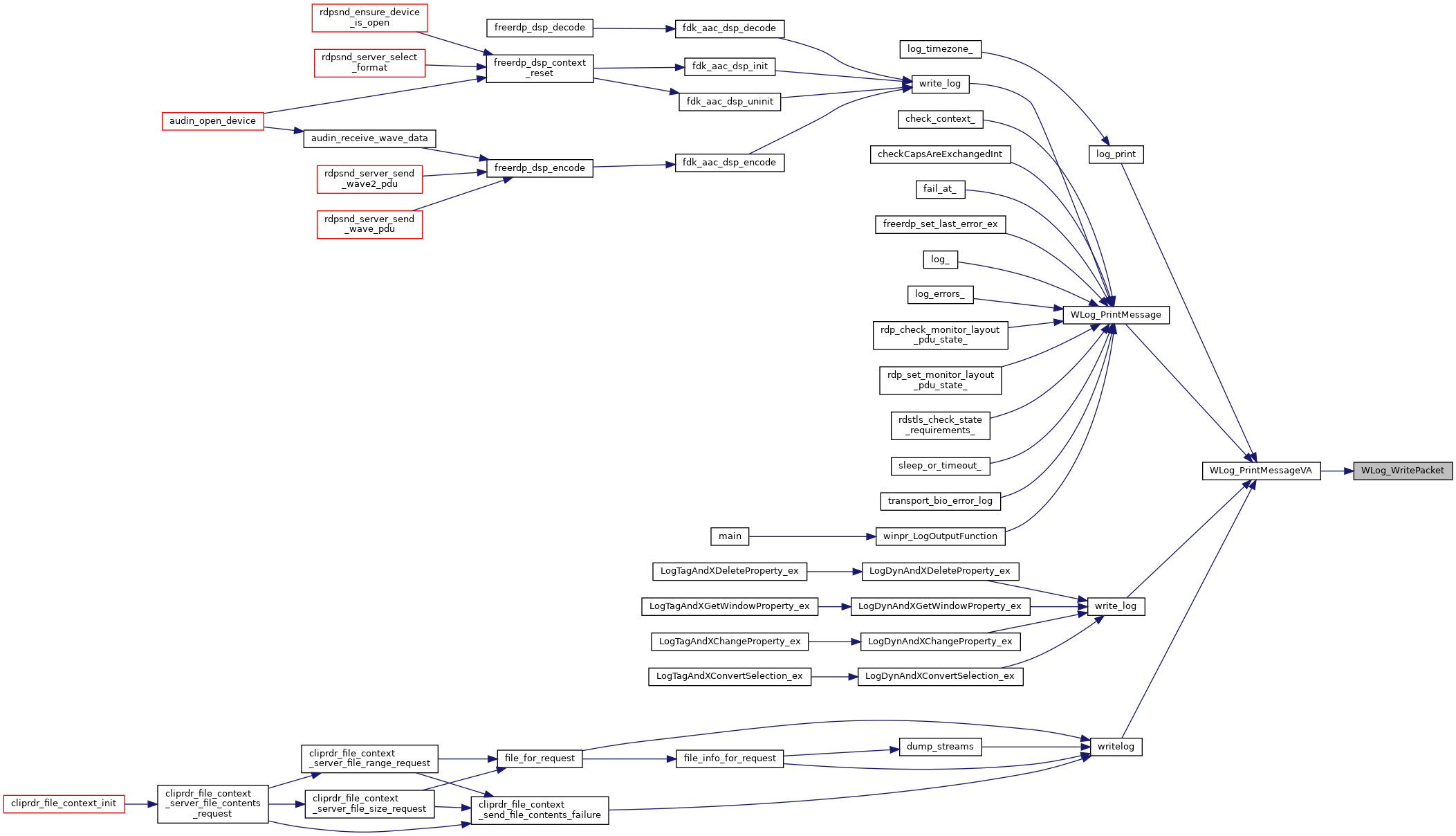static

## ◆ g_FilterCount

 DWORD g_FilterCount = 0
static

## ◆ g_Filters

 wLogFilter* g_Filters = NULL
static

## ◆ g_RootLog

 wLog* g_RootLog = NULL
static

## ◆ WLOG_LEVELS

 LPCSTR WLOG_LEVELS = { "TRACE", "DEBUG", "INFO", "WARN", "ERROR", "FATAL", "OFF" }

References for general logging concepts:

Short introduction to log4j: http://logging.apache.org/log4j/1.2/manual.html

logging - Logging facility for Python: http://docs.python.org/2/library/logging.html